## A Chess Puzzle, Part VII Episode 2 : The Rational Super QueenPart VII Episode 2 of A Mathematical Chess Puzzle:

We are nearing the end, but still there is the tallest mountain to climb. We need to prove the SUPER QUEEN THEOREM, which says that not only is there a chess position $Q$ to the unguarded queen problem on the unit continuum board $B_c$ (where $c$ are is understood as all reals in the open unit interval (0,1)), but that in fact that solution satisfies the Super Queen property — which is to say, that not only is there exactly one queen on every rank and file (“Rook Maximal”), but that there is also exactly one queen on every diagonal (“Bishop maximal”). This is not possible on finite boards because we run out of places on diagonals to put the queens that don’t attack an existing queen by a rook move.

Note: we exclude the endpoints 0 and 1 from the intervals to remove the “degenerate” diagonals on the corners, and so ensure that all rows, columns and diagonals are of positive linear measure. If they were included, then only one of the two degenerate diagonals through (0,0) and (1,1) respectively could be admitted and the “super queen” property would not hold.

To prove this theorem, as mentioned in the previous post, we will need to use a technique called Transfinite Induction. This is a concept which extends a more conventional technique called Mathematical Induction. The general idea of regular induction in mathematics can be expressed using the metaphor of a ladder:

Mathematical Induction: If you can prove that

1. you can get on the first rung of a ladder, and
2. you can also prove for any N that
if you can get to rung $N$,
then you can also get to the next rung $N+1$,

then you have proven you can go all the way up the ladder.

This does not seem very deep, and in fact seems obvious, but it is a technique used in many parts of mathematics. For example, consider the theorem:

THEOREM: For every integer $n \ge 1$, the odd sum  $1 + 3 + 5 … (2n-1)$ is a square, and in fact

$$S_n = 1 + 3 + 5 … (2n-1) = n^2\tag{**}$$

For example, for $n=1$, $1 = 1^2$, for $n=2$, $1 + 3 = 4 = 2^2$, and so on.

PROOF: We shall use (regular) mathematical induction. The first step is to prove we can get on the “first rung”, so we look at $n=1$ and can see that indeed $1 = 1^2 = (2n-1)^2$. For the next step, suppose that formula (**) holds for a specific $n = N$, that is, for a specific $N$ we have:

$$S_N = 1 + 3 + 5 … (2N-1) = N^2\tag{***}$$

We need to show that (**) also holds for $n= N+1$. We can write

$$S_{N+1} = 1 + 3 + 5 … (2(N+1)-1)^2$$

$$= 1 + 3 + 5 … + (2N -1)^2 + (2(N+1)-1)^2$$

which by definition can be rewritten:

$$= S_N + (2(N+1)-1)^2$$

and by the assumption (***) we have

$$= N^2 + (2(N+1)-1)^2$$

which simplifies to

$$= N^2 + 2N + 1 = (N+1)^2$$

which is formula (**) with $N+1$ substituted for $n$. We have just proved the second part of induction, which is that if (**) is true for $N$ then it is also true for $N+1$, and so the theorem is proved. QED.

### The Rational Super Queen

As a first step toward proving the Super Queen Theorem on the transfinite board $B_c$, we will first prove the analogous theorem on the rational unit board $B_{\mathbb{Q}}$, where $\mathbb{Q}$ is understood as the set of all rational numbers between 0 and 1 exclusive.

Referring back to the previous article on the Rook, both the rational unit board and the omega board have a countable infinity of “squares”. And as in that case, what makes the situations different is that the omega board (whose coordinates are integers) has a discrete topology, while the rational numbers are dense, and no square has a unique square “next to” it.

It should be noted that when restricted to just rational points, the concept of rook and bishop lines restricted to $B_{\mathbb{Q}}$ makes sense. The reason is that for any two “rational” points $p,q \in B_{\mathbb{Q}}$,, and any two rook or bishop attack lines $\lambda_p,\lambda_q$ which pass through $p$ and $q$ respectively, that their intersection is either empty or another rational point $r \in B_{\mathbb{Q}}$. That is, you will never have two points on the board whose common points of attack are irrational points outside of $B_{\mathbb{Q}}$. (Proof left to the reader)

Let us define $\Lambda$ to be the set of all rook and bishops lines (ie, all Queen lines) in $B_{\mathbb{Q}}$. Then we have

The Rational Super Queen Theorem: There exists a queen position $Q$ on the rational unit board $B_{\mathbb{Q}}$, such that for every queen line $\lambda \in \Lambda$ the intersection $\lambda \cap Q$ has exactly one point $q \in B_{\mathbb{Q}}$. That is, every Queen attack line in $B_{\mathbb{Q}}$ is occupied by exactly one queen in the position $Q$.

We will prove this theorem by (regular) mathematical induction. To do this we will need some definitions and to prove some  lemmas first.

Definition: a set $S$ is Well Ordered if there is an operator (which we will call $\le$), such that for all $x,y,z \in S$ we have

1.  $x \le x$
2.  Either $x \le y$ or $y \le x$
3.  if $x \le y$ and $y \le z$ then $x \le z$
4.  if $x \le y$ and $y \le x$ then $x = y$
5.  (Well Ordering): For any subset $T \subset S$, there exists a “smallest” element $x \in T$, ie, so that $x \le y$ for all $y \in T$.

The first four conditions describe “total ordering”, while the fifth (important) condition is what makes a “well ordering”. The principle of Mathematical induction is equivalent to the statement that the natural numbers $\mathbb{N}$ is well ordered.

Example 1: The positive integers $\mathbb{N}$ are well-ordered with the usual numeric ordering $\le$.

Example 2: The set of all integers $\mathbb{Z}$ is NOT well-ordered under $\le$, because $\mathbb{Z}$ itself is a set having no smallest value (violating condition 5).

Theorem 1:  The rational points $(x,y) \in B_{\mathbb{Q}}$ have a well ordering (but not the usual one $\le$).

Proof: It is known that the positive rational numbers $\mathbb{Q}$ are countable; that is, that there exists a function mapping the natural numbers $\{1,2,3,…\}$ to $\mathbb{Q}$ in a 1-to-1 way. To see that, observe that they can all be represented as fractions $p/q$ in a square array whose x and y coordinates indicate the numerator and denominator (below). Note that fractions that are not in simplest terms (e.g. 2/2) are skipped.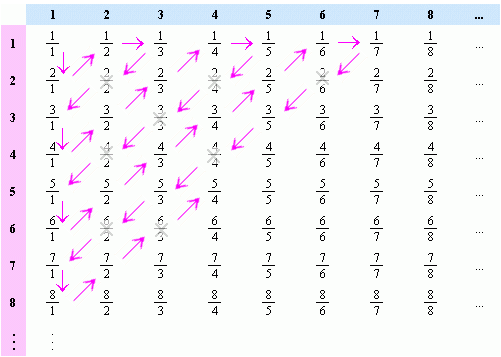As the arrows suggest, we can then “thread” the numbers onto a line, and number them by the order they appear, like this:

$$x_n = \frac{1}{1}, \frac{2}{1}, \frac{1}{2}, \frac{1}{3}, \frac{3}{1}, . . .$$

$$n = 1, 2, 3, 4, 5, . . .$$

This gives us a function $Q(n)$ which takes the integer $n$ to $x_n$. And so, we can define our new “ordering” (call it $\prec$) on the positive rationals by defining $x_n \prec x_m$ if and only if $n \le m$ in the usual sense. And this ordering is well-ordered, because for any subset $S$ of positive rationals, one simply considers the inverse set $S’ = Q^{-1}(S)$. SInce $S’$ is a subset of the positive integers, there must be a smallest one $m \in S’$, and by definition this is also the “smallest” in $S$, ordered by $\prec$.  QED

Theorem 3:  The rational points $(x,y) \in B_{\mathbb{Q}}$ have a well ordering.

Proof: We can follow a proof that parallels the one just given for Theorem 2. For we can use the inverse function $Q^{-1}(x)$ to map all rational points $(x,y)$ to non-negative integer points $(m,n)$, and by arranging them in a similar grid as above, “thread” the integer grid with a single integer line, and then use the inverse of this mapping to map the well-ordered integers to the rational points.  QED

Theorem 4:  The set $\Lambda$ of queen lines on the rational board is countable and can be well ordered.

Proof: Each (non-degenerate) queen line can be identified by a pair of distinct rational points $(x,y)$ and $(z,w)$ on the board which belong to it. The rational points are well ordered and (by the same arguments above), the pairs of such points also have a well-ordering.

Proof of The Rational Super Queen Theorem

Our goal is to prove that we can construct a position $Q = \{ q_0, q_1, . . . \}$ on the unit rational board, such that every queen line $\lambda \in \Lambda$ contains exactly one and only one element $q \in Q$.

By Theorem 4, the queen lines $\Lambda$ are countable. This means that we can assign an index $n$ to each of them which might as well be the integers 0,1,2…), which also gives them a well-ordering.

In addition, the points on the rational board itself is countable and has a well-ordering.

To prove the existence of a position $Q$ we shall use (regular) mathematical induction.

For step zero, we simply define $q_0 = b_0$ where $b_0$ is the first element in the well-ordering of the board. We also define $\Lambda_0$ to be the set of (four) queen lines which pass through $q_0$. Let us also define the set $Q_0 = \{ q_0 \}$.

For step 1, we consider the set of all queen lines which are not in $\Lambda_0$, and take the queen line $\lambda = \lambda_\alpha$ whose index $\alpha$ is the smallest among those. We then exclude from this $\lambda$ (considered as a set of points in the board) all points where it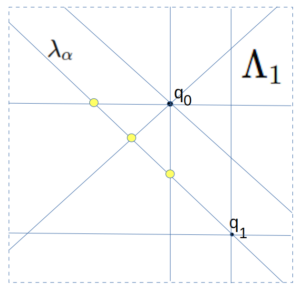intersects $\Lambda_0$ (shown here in yellow). This intersection is a finite set of points, and so we can pick for $q_1$ any other point on $\lambda$ not in this intersection. We then can define $\Lambda_1$ to be the set of all queen lines which pass through the set $Q_1 = \{ q_0, q_1 \}$. Note that $\Lambda_1 \supset \Lambda_0$ and is itself finite. We also note that any place where $\Lambda_0$ and $\Lambda_1$  share a point, those points are not in $Q_1$.

To generalize this last step inductively, for step $n+1$, we consider the set $S \subset \Lambda$ of all queen lines which are not in ${\Lambda_n}$, and among them choose a queen line $\lambda = \lambda_\alpha$ whose index $\alpha$ is the smallest within $S$. We can do this because ${\Lambda_n}$ is a finite set of queen lines, and so the remaining set is non-empty (in fact, countably infinite), and $\Lambda$ is well-ordered. We now consider the set of all intersections of $\lambda$ with lines in ${\Lambda_n}$. As before, this is a finite number of intersections and so we can choose for $q_{n+1}$ any point in the (infinite) queen line $\lambda$ not in this set, and define $\Lambda_{n+1}$ to be all queen lines passing through points $Q_{n+1} = Q_{n} \cup \{q_{n+1}\}$.

We now assert that (1) this tower of inclusive sets

$$\Lambda_0 \subset \Lambda_1 \subset \Lambda_2 . . .$$

is exhaustive, and its union will eventually include every single queen line $\lambda \in \Lambda$. In other words, the union is itself $\Lambda$. And furthermore, (2) the union of the tower of sets

$$Q_0 \subset Q_1 \subset Q_2 . . .$$

is our desired position $Q$ and that every member of $\Lambda$ meets $Q$ in exactly one point. The proof of this is below:

(1) To see that the $\Lambda_n$ tower is exhaustive, assume that this was not the case, and that there was some $\lambda$ that was missing from the tower, and so let us choose $\lambda_\beta$ to be the smallest of these missing ones in the well-ordering of $\Lambda$. But now consider how we constructed the set $\Lambda_{\beta + 1}$.  By construction, it was the union of $\Lambda_{\beta}$ with the  $\lambda_n$ with the smallest index $n$ which was not in $\Lambda_{\beta}$. But this means that $n$ must be greater than $\beta$, and so $\lambda_\beta$ should have been chosen at that step because it has a smaller index and is not in $\Lambda_{\beta}$.

(2) To see that every member $\lambda$ of $\Lambda$ meets $Q$ in at least one point, simply note that $\lambda$ must belong to $\Lambda_n$ for some smallest $n$ (since the union was exhaustive). But $\Lambda_n$ was defined to be all queen lines passing through $Q_n$, and so $\lambda$ must meet at least one element of $Q_n \subset Q$. But the points already chosen were selected from a set that excluded pairwise intersections of members of $Q_n$, and so $\lambda$ cannot possible meet any more than one element (as pairwise intersections were excluded). Hence $\lambda$ meets $Q_n$ at exactly one point, and by the construction, no later $Q_m$ sets meet $\lambda$ at all.

QED

This proof is remarkable, because nothing like it is possible with the finite boards. The thing which makes this work, is that at each step we are only removing a finite number of points from an infinite board, and so we can always find more in the remaining infinite sets to satisfy our conditions. Unlike the finite case, it did not matter whether any “queen line” was a rook or bishop style line. They were each treated equally in the set $\Lambda$ and the process of eliminating candidate points for the next step never exhausted the pool.

Having now proved the “Super Queen” solutions exists for the rational board, we are ready to pull out the big guns in the next post and deal with the Super Queen solution on the transfinite real board.

## A Chess Puzzle, Part VII Episode 1: The Fractal QueenPart VII Episode 1 of A Mathematical Chess Puzzle:

We have now come to the final (two-part) chapter of this two year journey, exploring the maximum “density” of various chess pieces on an infinite chess board, with the rule that no piece is attacking any other. The journey has taken us through many realms of mathematics, including geometry, set theory, group theory, differential calculus, real analysis, combinatorics, topology, measure theory, and transfinite numbers.

With that exhaustive list of mathematics, one would think that our last chess piece —- the Queen —- would have little more to offer us. After all, in terms of attacks, the queen is simply a Rook that can also attack like a Bishop, and so any position of “friendly” queens is also a position of friendly rooks and/or Bishops. In terms of set theory, the set of unguarded queen positions on a board is the intersection of unguarded Bishop and Rook positions.

But as we shall see, the Queen takes us on one final trip, through some of the deepest mathematics that we have yet encountered.

### Unguarded Queens on the Countable Board

There is one catch with the finite $N \times N$ board $B_N$, which is that while a maximal unguarded Rook  position consists of $N$ rooks, a maximal unguarded Bishop position will have $2N-2$ bishops, and so (on the finite boards at least) maximal unguarded queen solutions will not be maximal as bishops, merely “Rook maximal” (to coin a phrase).

There is a simple way in which one can take finite solutions to the unguarded queen problem and turn them into solutions on larger boards, and by extension to the countably infinite board $B_\omega$. We we show one such construction here.

For example, let’s start with a solution to the unguarded Queen problem on the 5-board $B_5$:

If we now go to the 25-board $B_{25}$, we can think of it as a $5 \times 5$ array of $B_5$ boards. So suppose we place one of the above solutions on each of the array locations corresponding to the queen solutions, like this:

it turns out that this is a Rook-maximal unguarded Queen position on the 25 board. It is not obvious that it is an unguarded Bishop position; even though it is clearly an unguarded rook position you have to verify that none of the queens in one block attack diagonally any other queens in the other blocks. Amazingly, none of them do. To see this, we only have to consider the diagonal attacks between the “blocks” of queens. As can be seen, when two such blocks are placed adjacent to each other, the queens in each block control separate diagonals from the other block:

In the exact same manner one can take this solution on $B_{25}$ and place five copies on a $B_{125}$ board to produce an unguarded queen solution there, and continue in with all larger boards that are powers of 5.

One can then extend this generally to the countably infinite board  $B_{\omega}$ by simply noting that each finite solution $Q_i$ contains all smaller solutions, and so you can simply take the union of all of them to cover $B_{\omega}$, that is. our position $Q_{\omega}$  is given by:

$$Q_{\omega} = \bigcup^{\infty}_{i=0} Q_i$$

To make this infinite solution explicit, we need to give for each horizontal integer coordinate $x = \{0,1,2 …\}$ the vertical location $y = \phi(x)$ where the queen in that column is located. There is a neat trick for doing this. We first explictly define the first five $y$ values of $\phi$ from $x=0$ to $x=4$:
$$\begin{cases} \phi(0) = 0 \\ \phi(1) = 2 \\ \phi(2) = 4 \\ \phi(3) = 1 \\ \phi(4) = 3 \\ \end{cases}\tag{PHI}$$
Note that $\phi$ is a permutation of the five digits $\{0,1,2,3,4\}$. We then define $\phi(x)$ for all other positive integers $x$ by writing its value in base 5, ie,
$$x_5 = d_1 d_2 … d_n,$$
where $d_i$ are base 5 digits, and then define
$$\phi(x)_5 = \phi(d_1) \phi(d_2) … \phi(d_n)$$
That is, we permute each of the input digits of $x$ by $\phi$ to get the base 5 representation of the output $y$. Note that this also shows that all rows contain a queen, because to find the column $x$ where a queen resides on row $y$, just write $y$ in base 5 and apply the inverse of $\phi$ on the digits to get $x$.

A close approximation of the infinite board $B_{\omega}$ solution can be seen below, with a scaled down version of the solution on the very large board $B_{625}$:

We should remark here that there is nothing magical about the number 5. We could just as easily have found another solution for the omega board using a $7 \times 7$ board queen solution as a template, such as this:

However, this approach does not always work. Not all 7-board solutions work for example, and no queen solutions on the 4-board work:

### The Troublesome Transfinite Queen

As with the Rook and Bishop, we now turn to unguarded queen problem on the board of the continuum $B_c$. Unlike the omega board whose “squares” are located at integer coordinate 0,1,2…, this board is transfinite, and has an uncountably infinite number of “squares” whose coordinates are real numbers. As with the Rook and Bishop, we will look strictly at the open bounded case where the board is the unit square (technically, that would be $B_I$, where $I$ is the open unit interval $I = (0,1) = \{ x: 0 \lt x\lt 1\}$. We have selected the open interval to eliminate the corner points and boundary.

As a naïve first attempt at constructing a solution, we might try the same trick as above, with an iterative process. The difference here, however, is that instead of a union of successively larger sets of discrete queens on the integer board, we create an infinite sequence of shrinking compact nested sets $Q_i, i=0,1,2,…$ where $Q_0 \supset Q_1 \supset Q_2 …$ and whose intersection forms the solution $Q$, that is,

$$Q = \bigcap^{\infty}_{i=0} Q_i$$

The first three iterations are shown below: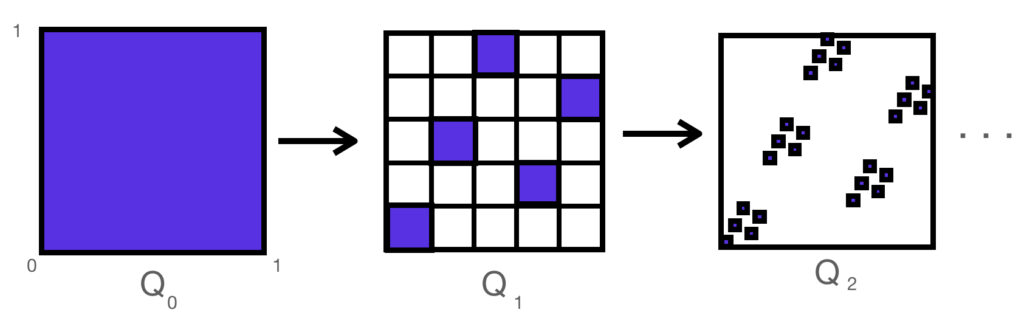And the resulting fractal set $Q$ looks very much like the board $B_{625}$ shown above. It can be shown that this set has a fractal dimension of 1, and a 1-dimension fractal measure of $\sqrt 2$

Now one would think that this “solution” $Q$ works, because at each iteration the cells do not “attack” each other as Queens. However, the actual “squares” on this transfinite board are points, so we need to make sure that there are no collisions in he limiting case.

### Failure!

… In fact, the solution $Q$ we have constructed is a failure.

Let’s first try to characterize the point set $Q$ mathematically, as we did in the case of the omega board.

In the omega board, whose squares had integer coordinates going off to infinity, we represented the values in Base 5, that is, multiples of powers of 5. In the case of the unit square, all coordinates are real numbers between 0 and 1, and we are dividing them by powers of 5.

We have the following theorem:

THEOREM: a point $(x,y) \in B_c$ is in $Q$ if and only if $x$ can be expressed as a Base 5 decimal, ie

$$x_5 = 0.d_1d_2d_3 ….$$

in such a way that the value $y$ can be expressed in Base 5 as

$$y_5 =0.\phi(d_1)\phi(d_2)\phi(d_3) …$$

And $\phi$ is the permutation on the digits $\{0,1,2,3,4\}$ defined by equation (PHI).

PROOF: The proof consists of observing that on the $nth$ iterative step $Q_n$ the points $(x,y)$ which are removed are exactly those where in their Base 5 representation the $nth$ digit of $y$ is not equal to $\phi(d_n)$. Conversely, if the $\phi()$ relationship holds for all digits of $x$ and $y$, then no iteration of $Q_n$ will eliminate the point $(x,y)$. QED

So if this is all good, what is the problem?

The problem is, there are many real numbers which can be expressed as infinite Base 5 decimals in two ways. In fact, any number whose Base 5 expansion terminates (ie, ends with 000…) has this property. For example, the (Base 10) value $x=0.2$ can be expressed (in Base 5) as either

$$x_5 = 0.100000000….$$

or

$$x_5 = 0.044444444….$$

(In the same way that in base ten 0.9999… = 1).

But if we apply $\phi()$ to these two decimal expansions we get two distinct values for $y$, ie

$$y_5 = 0.200000…$$

and

$$y_5 = 0.0333333…$$

…with the result that we have two distinct queens in the position $Q$ sharing the same $x$  value:

$$(x,y)_5 = (0.1, 0.2)_5 = (\frac{1}{5},\frac{2}{5})$$

and

$$(x,y)_5 = (0.0444…, 0.0333…)_5 = (\frac{1}{5},\frac{3}{20})$$

The two points can be seen here, along with (in red) many other queens in $Q$ which are mutally attacking, both by rook and by bishop moves:

And so we see that $Q$ is a total failure. Not only are there many members of this position that are hostile, but there are an uncountably many such failures!

As with the Rook and Bishop, the root difference between $B_{\omega}$ and $B_c$ has to do with topology. In the omega board, though infinite, it has a discrete topology with no limit points. In all of the cases with this continuum board, the queens which are attacking are limit points, belonging to members of the $Q_i$ sequence which bound the (rook or bishop) attack line from opposite sides of the line.

### An Unsolved Problem

Although I have tried a number of variations on this “fractal” approach, I have not found any explicit (fractal or otherwise) constructions of a queen position on the continuum board $B_c$ which is maximal unguarded one. That is, a set of points $Q \subset B_c$ defined by a closed-form computable function, such that for every horizontal and vertical line $\lambda$ intersecting $B_c$, there is exactly one point in $\lambda \cap Q$, and for every diagonal line, there is no more than one point in the intersection.

### A Solved Problem

As with many such questions about exotic properties of infinite point sets, it possible that no such closed form solution may even exist for the unguarded queens on the continuum board.

For example, a very similar problem to the unguarded queens is about the existence of “Two Point Sets”. That problem has been solved and can be stated like this:

TWO POINT SET THEOREM: There exists a set of points $T \subset \mathbb{R}^2$ such that every line in the plane contains exactly two distinct points of $T$.

This is a tantalizing statement, as it is about points and lines in the plane which satisfy a rule about which lines contains which points. And as with our unguarded queen problem, the quirky aspect of this (proven) theorem is that it only shows that a solution exists, but does not give any clue as to what it would look like.

In order to prove a theorem like this, however, requires some rather exotic techniques which depend on some deep hypotheses in set theory such as the Axiom of Choice and others. In particular, what is needed is a technique called Transfinite Induction.

This is a mathematical tool of which I had not been familiar during my younger days, and which most folks would have difficulty understanding at all, given that the word “transfinite” itself starts with Cantor’s discovery that there are sets which are so large that they are “uncountable” by the infinite set of numbers 1,2,3 etc.

### The Theorem

Using the techniques mentioned above, it turns out that not only will we be able to prove the existence of an unguarded queen solution as defined above, but an even stronger result, which has no equivalent statement on either the finite boards or even the countably infinite omega board.

We first begin with a definition:

DEFINITION: a chess position $Q \subset B$, satisfies the Super Queen property on $B$ if every horizontal, vertical AND (45-degree) diagonal line $\lambda$ intersecting $B$ contains exactly one member of $Q$.

Note that this is property is called super, because the property is not satisfied by any queen positions on the finite or countably infinite chess boards. On those boards, a queen position can be rook-maximally guarded positions, but not Bishop-maximally guarded. There will always be diagonals without queens controlling them.

The theorem we will prove in the next (and final) post on the topic will be the following:

SUPER QUEEN THEOREM: On the open continuum board $B = B_{I}$, where $I = (0,1) = \{ x: 0 \lt x\lt 1\}$, there exists a queen position $Q$ having the Super Queen property.

In other words, there is a position $Q$ on the board such that for every horizontal, vertical and diagonal line that intersects the board $B_{I}$, there is exactly one Queen on that line that belongs to $Q$ !

Stay tuned.

## A Chess Puzzle, Part VI: The BishopPart VI of A Mathematical Chess Puzzle:

We are now approaching the end of the story, with the final tale of the Fractal Queen approaching. But for now we must deal with with the Bishop, a close cousin to the Rook.

Recall that our puzzle revolves around the maximum “unguarded” positions of a chess piece on a board; in this case Bishops. In some sense, the Bishop can be thought of a Rook turned sideways, because both pieces can only move in two orthogonal directions, but the Bishop’s directions are along the 45 degree diagonals. The difference this makes though is that Bishops have a “color” while Rooks do not. Rooks can move to any square on the board while a Bishop on black squares can only attack other black squares.

This limited ability to only attack half of the squares that a Rook can, makes Bishops slightly “weaker”, and so it should not be surprise (as we showed in part I), that on an $n \times n$ board, we can place $2n – 2$ Bishops, giving them a density of roughly $\frac{2}{n}$ for large $n$, and asymptotically going to zero on the infinite board. So in some sense the friendly Bishop’s density on the board is roughly twice that of the slightly more powerful Rook.

But as with the rook, this is the two-dimensional density, while the unguarded Bishop positions clearly have a fractal dimension of one on the board. And as we saw with the Rook, once we go to the unit square board $\mathbb{B}_I$, with a continuum of “squares” at each real coordinate, the rigid shape of the solutions can loosen, so that instead of just the diagonal set with linear measure of $\sqrt{2}$ we can have a set of “friendly” Rooks on the board with 1-dimensional content of nearly 2:

and so, turning everything “sideways”, we can see that instead of a set of Bishops on the top and bottom row of the board with measure 2, we can stretch them toward each other, approaching a linear measure of almost $2\sqrt{2}$:

(Note that we have placed black and white circles in the corners to indicate that only the bottom corners are occupied with Bishops, which are in turn attacking the top corner locations). Again, as with the Rook solutions, there are no analogous version of these “continuum” solutions in the finite/discrete boards, as their topology is not dense.

Now one would think that the remaining piece — the Queen — would not be much different from the Rook or Bishop, as it simply has the capturing power of both. But as we will see, the problem suddenly become *very* different, and goes into some very deep and fascinating mathematics on the infinite boards.

## A Chess Puzzle, Part V: Rooks and TopologyPart V of A Mathematical Chess Puzzle:

### Review

We — that is, I — have been studying the (classic) “unguarded” chess puzzle, which is to say, how to pack as many of one chess piece on a chess board without any of the pieces attacking any others. But with the twist, that the board may be infinite, and possibly uncountably infinite. In the last post we saw that the set of maximal unguarded solutions for the Rook on the board $B_A$ (for any set $A$) had an interesting mathematical property, that they could be represented as the permutation group on the set $A$. In later posts we will see that the Queens also have some interesting properties, some of which can already be seen in our current focus on the Rook.

### What is Topology and Why does it Matter?

The glib definition of topology is “rubber sheet geometry”, which is to say, the study of what properties are preserved in an object even if they are made of rubber and can be twisted and stretched but not “torn”. For example, a solid ball and a solid cube can be deformed into each other just by stretching, but to deform a ball into a 1-holed doughnut you would need to puncture the ball to make the hole — which is not allowed.

One way in which a “topology” can be defined on a space is by specifying the way in which two points are “close” to each other, or whether two points can be “connected” by a continuous path.

We have been defining “chess boards” as two-dimensional things (denoted $B_A$) whose “squares” are identified with coordinates (x,y), where the x and y are in some subset $A$ of the real line $\mathbb{R}$. For example, the standard 8×8 chessboard we called $B_8$ uses the coordinates in the subset $\mathbb{Z}_8 = \{ 0, 1, 2, … 7 \}$ (though we could just as easily have taken {1, … 8} ). Among the other coordinate sets $A$ we could consider are the whole numbers, the rationals, the algebraic numbers, and the non-negative real numbers, or for that matter the whole real line.

### Topological Density

To see why questions about chess puzzles on various boards $B_A$ may have different answers depending on topology, consider that one of the properties of a subset of $\mathbb{R}$ is called density. A set $A \subseteq \mathbb{R}$ is said to be dense at a point $x \in A$ if for any other point $y \in A$ where $y \neq x$, there exists another point $z \in A$ strictly between them, ie, such that $(z-x)*(z-y) < 0$. The set A is everywhere dense if it is dense at every point of A, and nowhere dense (also called “discrete”) if it is not dense at any point of A.

For example, the whole numbers $\mathbb{W}$ is nowhere dense because there are no other integers between n and n+1. Meanwhile, the rationals $\mathbb{Q}$ are dense, because if $p$ and $q$ are two distinct rational numbers (ie, can be expressed as an integer fraction $m/n$), then it can be shown that $(p+q)/2$ is another rational strictly between them.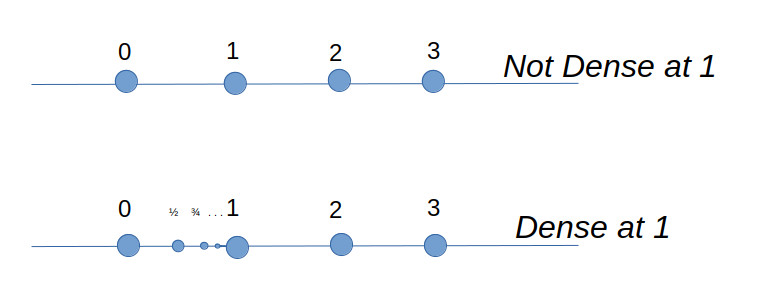And so, to see the reason why density is relevant for chess, simply note that the moves and attack regions for both the King, Pawn and Knight are defined by the squares *adjacent* to their current location, and on a board $B_A$ where $A$ is everywhere dense, there is no such thing as an adjacent square, because there is always another square in A in-between. In other words, the “unguarded” puzzle for Kings, Pawns and Knights does not make sense for dense sets $A$ such as rationals, algebraics, or reals. Meanwhile, because the attacks of Bishops, Rooks, and Queens is defined in terms of directions on the board (horizontal, vertical, diagonal), they have well-defined meanings even on everywhere dense boards.

### Connectedness

Strictly speaking, a set is topologically connected if it cannot be represented by a union of two disjoint sets. By that definition, a set like the integers or the rationals is nowhere connected. For example, the rationals can be represented as $\mathbb{Q} = \mathbb{Q}^+ \cup \mathbb{Q}^-$, where $\mathbb{Q}^+$ and $\mathbb{Q}^-$ are the sets of rationals greater and less than $\sqrt{2}$, respectively. These are both open sets because for any rational number $x \in \mathbb{Q}^+$, all other rationals (sufficiently close) to $x$ are also in the same set.

Nevertheless, we can still define “weaker” forms of connectedness on the rationals, in the sense that the closure of $\mathbb{Q} \subset \mathbb{R}$ is connected. There are also other more general definitions. A set is considered connected, intuitively, if you can get from any place in the set to any other by a “connected” path lying wholly within the set (whose definition needs to be made clear).

For example, suppose we take the set of whole numbers $\mathbb{W}$, and define that two elements $m, n \in \mathbb{W}$ to be “weakly connected” if they differ by no more than 1. So, 2 is connected to 3 and 1, as well as itself, but 2 is not connected to 4. More generally, we can say that two subsets A and B of $\mathbb{W}$ are weakly connected to each other, if there exists a member a in A and b in B such that a and b are connected. Finally, we can define a single subset $A \subseteq \mathbb{W}$ to be weakly connected, if for any two elements $a,b \in A$, there exists a finite sequence of elements $\{ a_i \} \subset A$ such that $a = a_0, a_n = b$, and where $a_i$ and $a_{i+1}$ are connected for all $i = 0…n-1$.

With this definition, we can see that the set $A = \{0,1,2,3,4\}$ is a weakly connected set, but that $A=\{0,1,3,4\}$ is not connected, because there is no way to to get from 1 to 3 by connected elements in $A$ (2 is missing and forms a gap).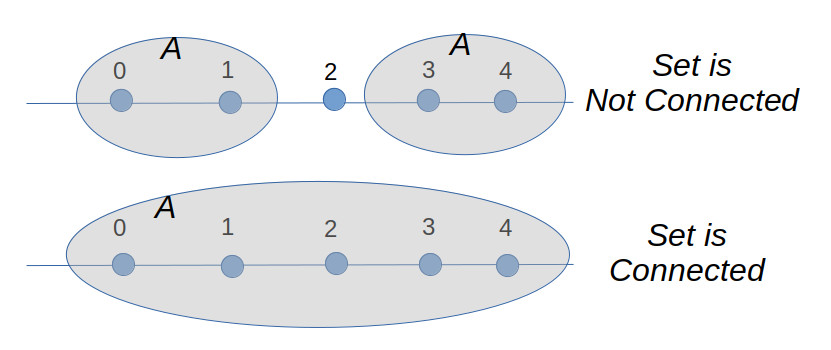The reason all this matters is that we will see that for the solutions to the unguarded rook problem, the topology of those solutions differs when we go from the infinite integer board $B_{\omega}$ to the infinite rational board $B_{\mathbb{Q^{+}}}$ or real board.

### The Topology of the Integer Board

Just as with the rationals, we can define “weakly connected” on the integer board $B_{\mathbb{W}}$, by first specifying that two squares a and b are “weakly connected” if they are a Kings move away from each other, that is, one square away horizontally, vertically, or diagonally. And by extension, we can say that any subset $A$ of $B_{\mathbb{W}}$, is connected, if any two members $a$ and $b$ of $A$ can reach each other, by a king moving strictly within the set $A$. From this point on, we will use “connected” to mean “weakly connected”, unless we say otherwise.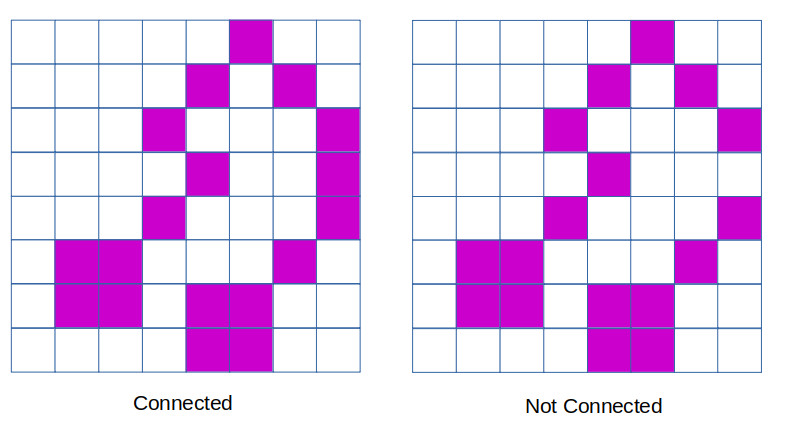Connected Rook Theorem 1: On any finite board $B_n$, the only connected maximal solutions to the unguarded rook problem are the two diagonal solutions, ie the graphs of the function $y=\phi(x)$, where

$$\phi(x) = x$$

or

$$\phi(x) = n – 1 -x$$

We first claim that if two squares in an unguarded rook solution are both connected to a third one, then all three must lie on the same diagonal (northwest or northeast). For, consider the case where square $a$ is connected to square $b$ along the north-east diagonal. We can go through and eliminate with an x all of the squares which are attacked by either $a$ or $b$. And what we see is that the only remaining square which a third rook can be placed and still be attached to $a$ is the lower-left corner.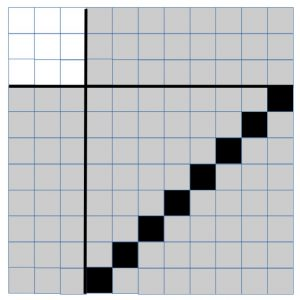By repeated application of this claim, what we have just shown is that all of the rooks in a connected component of an unguarded rook solution must lie on the same diagonal. Since this is a maximal unguarded solution, there must be a rook on the first row somewhere. We now claim that it must either be on the leftmost square (forming the northeastern diagonal solution), or else the rightmost square (forming the northwestern diagonal solution). For suppose that the rook is anywhere else along the bottom row, and that (without loss of generality) that it is part of a northeasterly diagonal.

Once again, by a process of eliminating all other squares under attack by the northeasterly diagonal line of rooks, we can see that this leaves a disconnected square in the upper left corner as the only available locations of the rooks that must occupy the remaining rows and columns. But none of these squares are connected to the northeast diagonal, and so a maximal unguarded solution cannot be constructed in this manner that is also connected.

QED

Connected Rook Theorem 2: On the infinite integer board $B_{\omega}$, the only connected maximal solution to the unguarded rook problem is the main diagonal solution, $\phi(x) = x$.

Proof: Clearly the above solution is maximal. Suppose that there is another maximal solution where there is a rook located at a position $P = (m,n)$, where (without loss of generality) we can assume $m > n > 0$. By eliminating all cells attacked by this rook, we now have a board segmented into four non-empty regions, (I, II, III, IV),  each of which would be disconnected from each of the others, save for their connection to the rook at $P$.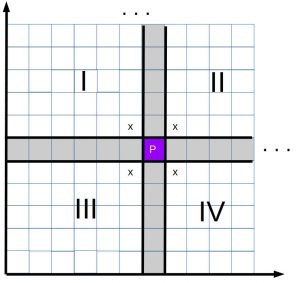By the same argument previously, either $P$ connects regions I to IV by the north-west diagonals, or else it connects regions II and III, by the northeast diagonals. If we choose to join regions II and III, then region III (including P) is an $m x n$ rectangle, in which we would have to fit $m$ rooks, even though we only have $n$ rows. This would force at least two rooks to occupy the same rows, which is not allowed. This leaves us with P joining up regions I and IV, by a northwest diagonal.  Now region I only has $m-1$ columns, while region IV has only $n-1$ rows, and so we have only a finite number of additional rooks we can add to this solution (region II not being connected to P). Thus neither option allows us to define a maximal solution to the unguarded rook problem, meaning that we must conclude that only the case where $m=n$ is valid.

QED.

### Unguarded Rooks on a Rational Board

While we could take the entire set $\mathbb{Q}$ of rational numbers, for our purposes it suffices to look at a much smaller but equivalent space, which is the unit interval of rational numbers, ie

$$\mathbb{Q}^* = \mathbb{Q} \cap [0,1]$$

The set of rationals is countably infinite, and so our board $B_{\mathbb{Q}^*}$ has the same number of “squares” as the infinite integer board. Nevertheless, the unguarded rook problem on this board has a surprising answer in store for us, given by the following theorem:

Connected Rook Theorem 3: There are an infinite number of connected maximal unguarded Rook solutions on the rational unit board $B_{\mathbb{Q}^*}$.

Comment: Just so you can see how on earth this could be possible, consider just one valid solution, defined on $\mathbb{Q}^*$ by the function:

$$\phi(x)= \begin{cases} \frac{x}{2}, & \text{if x \in [0, \frac{2}{3})} \\ 2x – 1, & \text{x \in [\frac{2}{3}, 1]} \end{cases}$$

The function $\phi(x)$ is a piecewise-linear function, which is a bijection on the set $\mathbb{Q}^*$.

The graph on $B_{\mathbb{Q}^*}$ shows that the placement of the rooks on the board looks like this: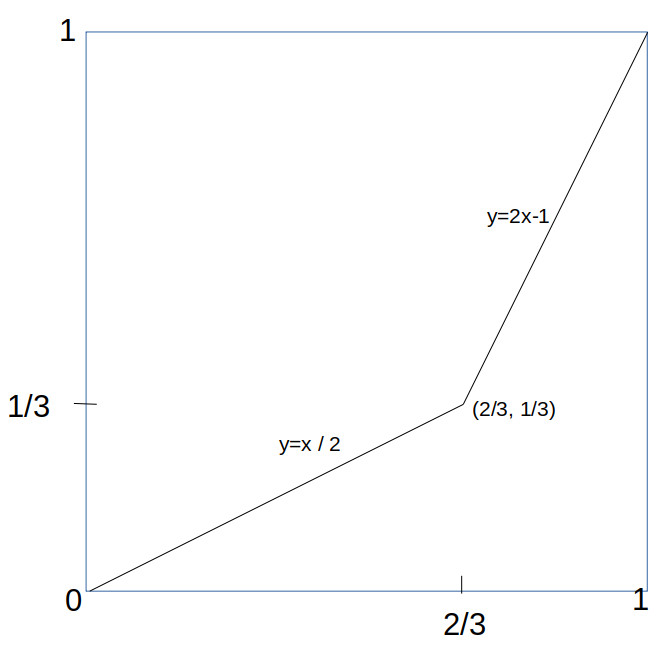what makes this work is that linear functions are invertible on the rational numbers, and that the rational numbers (unlike the integers) are *dense*. The same trick would not work on the integers, because you can’t apply y = x/2 to the odd integers and get another integer back.

Just to remind the reader of what this picture is about, that thin line is a chess position, consisting of a set of infinitely many rooks along that line, so an (infinite) magnification would reveal them, like this: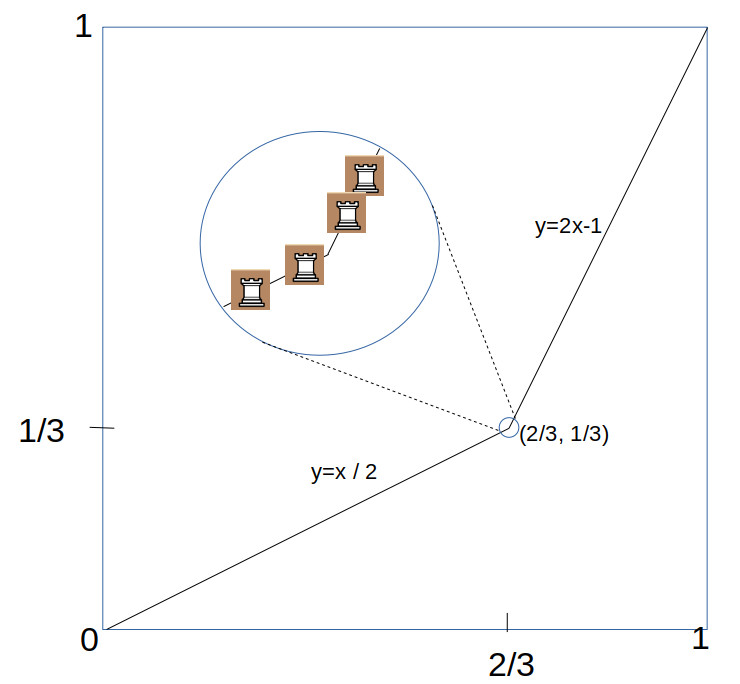This example is just one of many other such piecewise-linear functions which do the trick. The only requirement is that the functions must be strictly monotonic (increasing or descreasing) on the interval $\mathbb{Q}^*$, and (to ensure it is maximal), covers the entire interval, either by $\phi(0) = 0, \phi(1) = 1$ or else $\phi(0) = 1, \phi(1) = 0$.

It turns out that the number of such functions is infinite. This can be seen easily by just noting that there was nothing special about the bend at $x=2/3$, and you could create a similar function with a bend on any other rational number between zero and one, of which there are countably infinite many of them.

### Fractal Content

The (two-dimensional) density of the Rook solutions is zero, since lines have zero area. This is the case for all three pieces Rook, Bishop and Queen. But we can still compare the relative density of each of these pieces, by measuring the (one-dimensional) “fractal” content of the positions. For example, the fractal content of the solution shown above is simply the length of the two line segments, which is $2\sqrt{5}/3 \approx 0.75$. But we can actually do much better, by pushing the “corner” of the two lines toward the bottom right like this: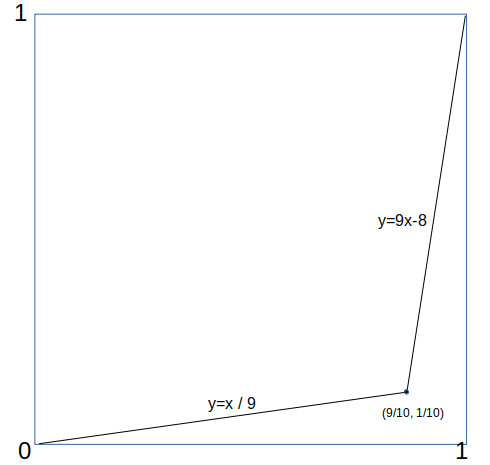in which case the fractal density is $\sqrt{82}/10 \approx 1.82$. As can be seen, by pushing further the rook solutions can attain fractal densities as close to 2 as we like.

### Uncountably Many Solutions

We showed above that there are an infinite number of connected maximal Rook solutions on the rational interval board $B_{\mathbb{Q}^*}$. But you can actually say a lot more; in fact the number of such solutions can be shown to be uncountably infinite, which is to say, there are at least as many of these solutions as there are real numbers (the infinity of the continuum) !

One way you can prove that the number of solutions is uncountable, is to construct a mapping between the (uncountable) set of all infinite decimals between 0 and 1, and a piecewise-linear function with rational coefficients, whose graph is a maximal connected solution of the unguarded Rook problem.

To do this, we first define for each digit from $i = 0 . . . 9$, a different piecewise linear function $f_i()$ on the unit square, simply by mapping the digits to different “bend locations” in the square (having rational coordinates):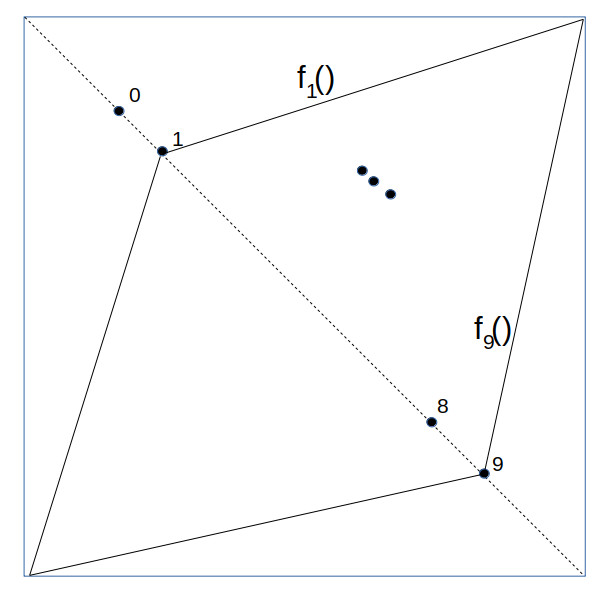Next, we take the unit square and along the main diagonal, lay out an infinite number of smaller squares, each half the size of the previous: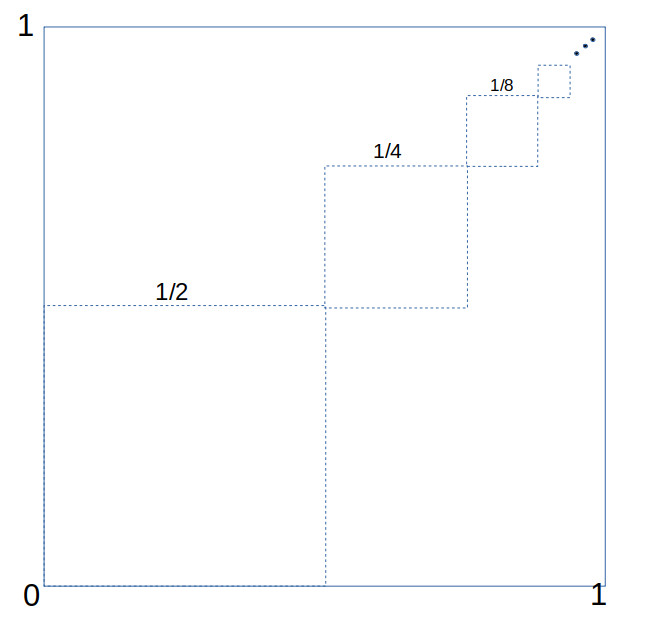We now show how to map each infinite decimal fraction $z$ (e.g. $z = 0.2739 . . .$ ) to a specific piecewise-linear function $F = F_z(x)$ on the unit square. For each of the smaller squares we have placed inside the unit square, we go through the decimal digits in $z$ and for each digit $d$ define the corresponding function $F_z(x)$ restricted to that range by $F_z(x) = f_d(x)$, corresponding to that digit $d$.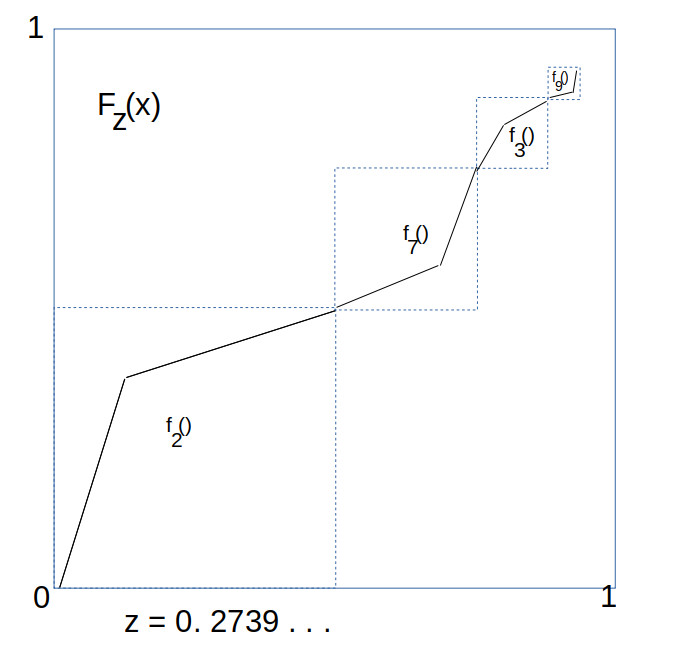As these infinite number of squares converge to the point $(1,1)$, we define $F_z(1) = 1$, which gives a complete definition on the whole unit interval. Since this mapping is injective, this means that the number of piecewise linear functions with rational coefficients is at least as large as the number of reals on the unit interval, which is uncountably infinite. This completes the proof.

So yes, there are a lot of them. And again, the reason this is so different from the integers has to do with the topological density of the sets.

Just to close the loop, if we look at the full real interval $I = [0,1] \subset \mathbb{R}$, it is actually much easier to construct topologically connected (in fact, compact) maximal unguarded Rook solutions. To construct such solutions, all that we require is that it be the graph of a function $y = \phi(x)$, where $\phi$ is a bijection on $[0,1]$ such that it is continuous and monotonically increasing or decreasing. That is, if $x > y$ then $\phi(x) > \phi(y)$. A simple set of examples of this are the powers of x, such as $\phi(x) = x^2, \phi(x) = x^3$, and so on.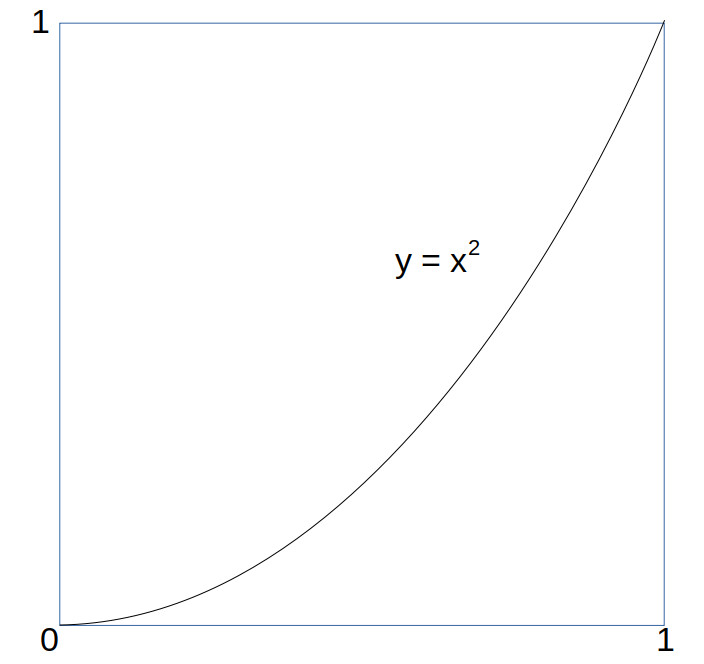As with the rational board case, with higher powers of x the curve begins to hug the bottom and right side of the board, with a curve length approaching 2. But can we do any better, or is 2 the largest 1 dimensional fractal content we can attain for connected unguarded rook solutions? The answer is given by the following theorem:

Fractal Content Rook Theorem: The path length of  a connected maximal unguarded Rook solution on the unit interval is not more than 2. That is, the length of any curve $y = \phi(x)$ on the unit interval $[0,1]$ where $\phi(x)$ is a continuous monotonically increasing function from 0 to 1 on the interval is less than or equal to 2.

Proof: We will need to use a little bit of calculus to do this. First, we parameterize the curve by $c(t) = (x(t),y(t))$ where the parameter $t$ ranges from 0 to 1, and

$$\begin{cases} x = x(t) = t \\ y = y(t) = \phi(x(t)) \end{cases}$$

From this we can compute the path length $s$ given by the integral (ie, sum):

$$s = \int_{t=0}^1 \frac{ds}{dt} dt$$

where $ds$ is the incremental increases in the path distance by $(dx,dy)$, given by

$$ds = \sqrt{dx^2 + dy^2}$$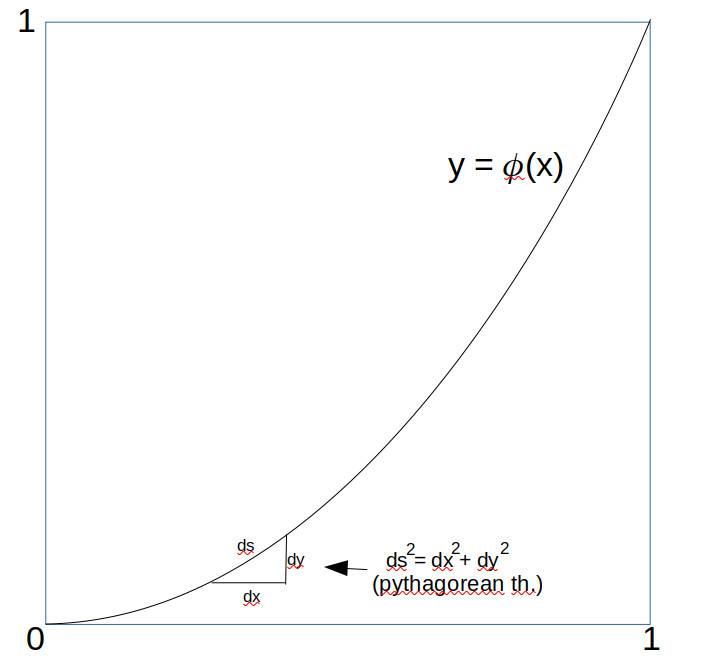For those unversed in calculus, think of $ds$ as just very small line segments into which the curve has been broken, and use Pythagorean Theorem). Now $dx/dt = 1$ since $x=t$, and $dy/dt \ge 0$, given that $y = \phi(x)$ is monotonically increasing. We can thus take advantage of the inequality

$$\sqrt{a^2 + b^2} \le \sqrt{a^2 +2ab + b^2} = (a + b)$$

when $a,b \ge 0$ to assert that (formally)

$$\frac{ds}{dt} = \sqrt{(\frac{dx}{dt})^2 + (\frac{dy}{dt})^2} \le \sqrt{(\frac{dx}{dt} + \frac{dy}{dt})^2} = \frac{dx}{dt} + \frac{dy}{dt}$$

and so we can conclude (since x(1) = 1 and y(1) = 1):

$$s \le \int_{t=0}^1 \frac{dx}{dt} + \int_{t=0}^1\frac{dy}{dt}dt = 1 + 1 = 2$$

which was to be proved. QED

We have now pretty much exhausted the study of unguarded Rooks, so it’s time to touch base briefly on the Bishop, before we move on (at last) to the unguarded Queens.

## A Chess Puzzle, Part IV: A Group of RooksPart IV of A Mathematical Chess Puzzle:

### Review

We have been studying the (classic) “unguarded” chess puzzle, which is to say, of packing as many of one chess piece on a chess board without any of the pieces attacking any others. What we have been doing is to measure the “density” $\delta$ of the pieces on the $n \times n$ chess board, as $n \rightarrow \infty$. So far we have shown that the densities for the Pawn, Knight and King are 1/2, 1/2, and 1/4 respectively, and also shown what such solutions look like on the infinite board.

In this post we will start to look at the other three pieces, Rooks, Bishops, and Queens, and will see how different things become. The reason things are different is that, unlike the Pawn, Knight and King, these remaining pieces have a “control region” which is unlimited, and extends out infinitely on an infinite board.

Our first clue that things are different may be seen by this analogous result from the previous post:

### The Chess Density Theorem – Rooks, Bishops and Queens

DENSITY THEOREM 2: For Rooks, Bishops and Queens, their asymptotic density $\delta$ on the infinite board ($B_{\omega}$) is given by:

$\delta = 0$.

In other words, on an infinite chess board, the fraction of squares you can cover with “friendly” Rooks, Bishops or Queens is vanishingly small. As a reminder, by “friendly” I mean that none of the pieces are threatening each other by standard chess rules. Also, if you were to throw a dart at the board with a densest packing of friendly pieces, the odds of hitting a nonempty square is zero.

PROOF: Unlike the Pawns, Knights and Kings, the proof of this theorem is practically a one liner, as we can easily show that for each piece X in the set {R,B,Q}, there is a constant C>0 so that if $\Phi \in \mathscr{U}(B_n,X)$ is an unguarded chess position of that piece on the n-Board, then its weight (ie, the number of pieces in the position) $\Vert \Phi \Vert$  is bounded by

$$\Vert \Phi \Vert \le C*n$$

And so the density $\delta_n$ on the n-board is bounded by

$$\delta_n = \frac{\Vert \Phi \Vert}{n^2} \le C/n$$

and so, since $C$ is a constant, then the quotient $C/n \rightarrow 0$ as $n \rightarrow \infty$.

For Rooks and Queens, the constant $C=1$ will do, because the n board has n rows, and neither rooks nor queens can share a row. For Bishops, the constant $C=2$ will do, because there are exactly $2n-1$ northwest diagonals on the n-board, and friendly bishops cannot share a diagonal. $\blacksquare$

Okay, so friendly Rooks, Bishops, and Queens have to be very sparse on the infinite board. But what do their solutions look like?

### The Rook

From part one we already know at least what one unguarded Rook solution looks like on the 8-board:

Which immediately suggests a general solution on the infinite board:

$$\Phi_R(x,y)= \begin{cases} R, & \text{if x = y} \\ \varnothing, & \text{otherwise} \end{cases}\tag{Rook}$$

On the infinite board the position looks like this (the diagonal line is infinitely thin):

We can readily see that this is indeed a solution, and it is also apparent that except for that infinitely thin line of rooks the board is empty. But are there any other solutions? There are, and they have a very interesting characteristic. At least for me…

DEFINITION: A chess position $\Phi$ on a board B is said to have the Rook Property if and only if every row and column of B has exactly one non-empty square.

Obviously, any maximally unguarded Rook positions have the Rook property. But this is also a property of maximally unguarded Queens, so the definition is a bit more general.

If you think about it, if a position $\Phi$ has the Rook Property on the n-Board, then this means for any integer $x$ between 0 and $n-1$, there is exactly one integer $y$ in that same range so that $\Phi(x,y)$ is a non-empty piece. In other words, $y$ is a function of $x$, so that we can write $y = \phi(x)$. And not only that, but since no two non-empty squares of $\Phi$ can have the same row (y-value), this means that if $\phi(x_1)= \phi(x_2)$, then $x_1= x_2$. In other words, the function $\phi(x)$ is also an invertible function (bijection) from the set {0,1,….n-1} to itself.

Such functions $\phi(x)$ have a more common name, which is that they are permutations (rearrangements) of the set of n elements. The set of all permutations form what is called the symmetry group Sym(n), and it appears all over the place in group theory.

A group is simply a set $G$, together with a “group product” often denoted $a{\circ}b$, with the property that (1) there is an “identity” element $e$ for which $e{\circ}a = a{\circ}e = a$ for all $a \in G$, (2) for every $a \in G$ there is an “inverse” $a’$, so that $a’{\circ}a = a{\circ}a’ = e$, and (3) the product is associative, ie $(a{\circ}b)\circ c= a{\circ}(b \circ c)$. The integers and addition are an example. Group theory has a lot of uses.

In any case, it can (easily) be proved that this relationship between $\Phi(x,y)$ and $\phi(x)$works both ways, on any board:

THE ROOK GROUP THEOREM: If $A$ is any set (finite or infinite), $\Phi$ is a position on the board $B_A$, then $\Phi$ has the Rook Property if and only if there is a permutation $\phi \in Sym(A)$ such that the position $\Phi$ satisfies the following:

$$\forall x,y \in A: \Phi(x,y) \neq \varnothing \text{ if and only if } y=\phi(x)$$

In particular, if consider only rook positions on a board $B_A$, then there is an exact one to one correspondence between the maximally unguarded positions and elements of the symmetry group $Sym(A)$. In other words, the set of maximal friendly Rook positions forms a group, and you can actually define a group operator (*) on solutions so that the group product of two such solutions forms another solution!

In fact, if you inspect the one example we showed for eight rooks on the standard 8-board, you can see that it corresponds to the identity element of Sym(8), ie, $\phi(x) = x$.

This may all sound like gobbledegook, but it gives us some simple ways to cook up new examples of friendly Rook positions. For the eight board, all we need to do is write down a couple of permutations of the set {0,1,…8}.

For example consider the following two permutations:

(3,2,1,0,7,6,5,4) and  (1,3,5,7,0,2,4,6).

These can be thought of as two functions, each being the ordered list of values you get by plugging in 0, then 1, then 2, and so on. The chess positions that correspond to them, are simply the graph of the functions on the board, like this:

To show how to “multiply” these two solutions to get a new one, you just compose their two permutations as functions. So for example, the first permutation takes 0 to 3, while the second permutations takes 3 to 7, so the composition of the two takes 0 to 7, and so on. We can show their group product graphically like this:

So how many solutions on the n-board are there? From our theorem above we know the answer, which is the same as the number of permutations of $n$ objects, which is to say $n! = 1*2*3*4*….*n$ (n factorial). For the 8×8 board, this works out to 8! = 40320 solutions.

Now how about $B_\omega$, the omega Board? How many solutions? We know from our theorem the friendly Rook positions correspond to the elements of the permutation group $Sym(\mathbb{W})$, where $\mathbb{W}=\{0,1,2,3,…\}$, the set of whole numbers. Now the set of whole numbers is infinite, and has cardinality $\aleph_0$, so is countably infinite. But how many permutations of this set are there?
We will have to do some transfinite arithmetic. To specify a permutation $\phi$ on $\mathbb{W}$ we have to chose values for $\phi(n)$, for every value of n. For n=0 we can pick any of an countably infinite ($\aleph_0$) number of values for $\phi(0)$. Suppose we pick 7. Now how about the next value for n=1? We can’t choose 7, but we still have an infinite number of choices left. And so on. Altogether, we have to make a (countably) infinite number of choices among a countably infinite number of values. This gives us a total count of

$$\aleph_{0} \times \aleph_{0} \times \aleph_{0} \times … =\aleph_{0}^{\aleph_0}$$

solutions. This is an uncountably infinite number of solutions, equal to c, the infinity of the continuum, the number of points on the real line.

Wow. That’s a lot of solutions.

As we will see later, the queen also has a similarly large collection of unguarded solutions, but the Rook is unique in being the only piece of the six whose solutions have a natural group structure.

In the later posts we’ll take a look at the Bishop and queen, and also start to examine fractals and even more infinite boards.

## A Chess Puzzle, Part III: The Pawns, Knights and KingsPart III of A Mathematical Chess Puzzle:

This is part III of my series of posts on the “Chess Density Problem”. In Part I, I introduced the problem and laid out some definitions to clarify what I meant by some of the terms such as “Board” and “Density”. In Part II I gave everyone a breather.  Hope you’ve rested up. In this one the math is going to be a bit thick, but the purpose of it all will be to prove this first theorem:

### The Chess Density Theorem – Pawn, Knight and King

DENSITY THEOREM 1: For Pawns, Knights, and Kings, their asymptotic density $\delta$ on the infinite board ($B_{\omega}$) is given by:

1.1) $\delta(P) = 1/2$.

1.2) $\delta(N) = 1/2$.

1.3) $\delta(K)= 1/4$.

In other words, on an infinite chess board, you can pack at most half of the squares with “friendly” Pawns or friendly Knights, and only a quarter of the board with friendly Kings. As a reminder, by “friendly” I mean that none of the pieces are threatening each other by standard chess rules.

### Plan of Attack

As a starting point, we can note that the solutions found on the standard 8-board can be seen as built up from simple “tile” patterns made of identical small boards: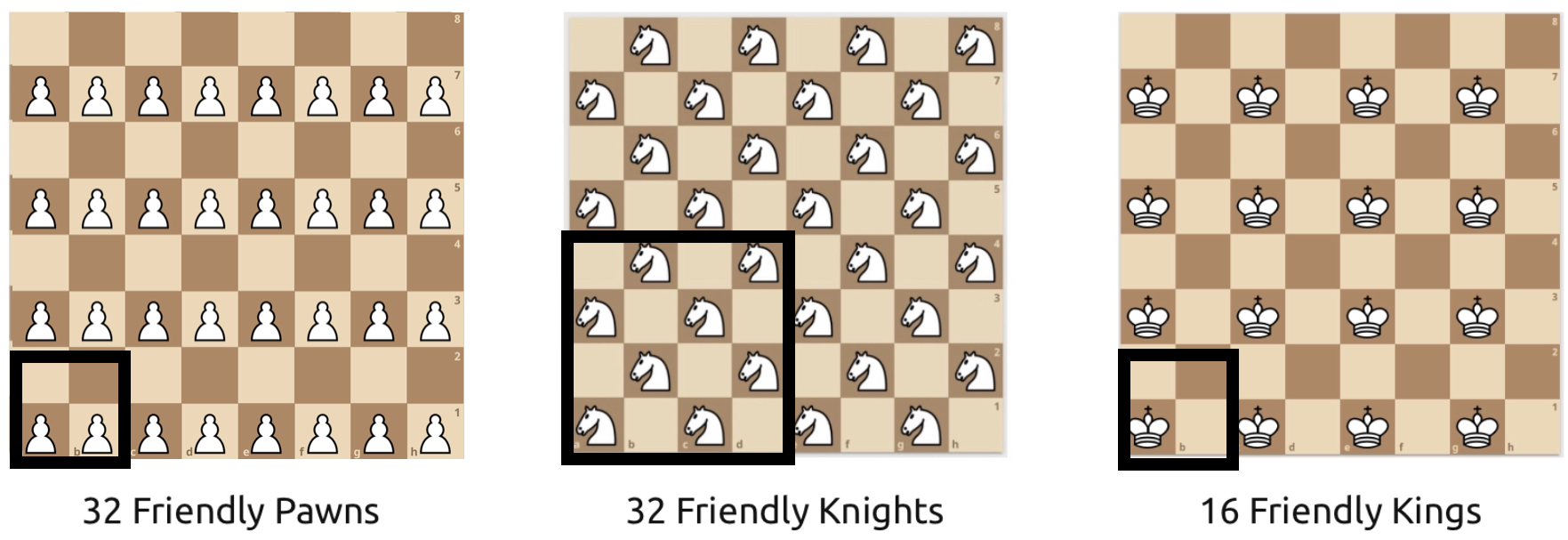These tiles each have the densities that are claimed by the Density Theorem above. If we can prove that no such tiles can have a greater density, we can show that this must hold for larger boards. The way which we will do this is to define a precise way to “measure” the density of any arbitrary block of squares, and prove some useful properties of that measure-function.

### Definitions

It always helps to define things precisely. There are a lot of fancy symbols, but the general ideas should be clear.

### The Pieces

The six standard Chess pieces are King, Queen, Bishop, Knight, Rook, and Pawn, which we will denote with the initials {K,Q,B,N,R,P}, respectively. To this set we will also add the “Empty” piece, denoted $\varnothing$, which indicates that a square is empty.

The set of all pieces including the empty piece will be denoted

$$\mathscr{P} = \{K,Q,B,N,R,P,\varnothing \}$$

We include that “empty” piece to allow us to explicitly specify that a square is empty.

### Control Zones

DEFINITION: The control zone of a piece X at position $x$ on a board B is the set of all other locations $y \in B$ which (by the standard rules of chess) are subject to attack by X (with no other pieces on board).

The Control Zones of the Pawn, Knight and King are shown in blue below:

One thing to note about these three pieces is that their control zones can be defined by a specific finite set of integer offsets from the location of the piece itself. The same cannot be said for the other three pieces (Rook, Bishop, Queen), as their Control Zones are not defined by a fixed set of offsets, but a set of directions on the board, by which they can attack (again, assuming that there are no other pieces blocking the way).

The Control Zone directions for the Rook, Bishop and Queen are shown below.

As we begin to work with the infinite boards we will see that this difference between the two sets of pieces profoundly affects how “dense” they can be.

In particular, on infinite boards the control zones of the Rook, Bishop and Queen are also infinite.

### Chess Positions

A lot of the definitions below are just stuff I made up. If they correspond to standard chess terms I will mark them with (standard). If I feel that the definition may not be clear, I will give an example as well.

DEFINITION: For a given board $B$ and any subset $\Omega \subseteq B$, a chess position $\Phi$ on $\Omega$ is simply a function $\Phi$ that for each location $x \in \Omega$ there is a piece $p \in \mathscr{P}$ given by $p = \Phi(x)$ at that location. That is, it is a function

$$\Phi: \Omega \rightarrow \mathscr{P}$$

For all of the chess positions we are considering for this puzzle, we only have one kind of piece on the board at the time. And so we will call them “Pawn-positions”, “Queen-positions” etc. Thus if $\Phi$ is a King-position on $\Omega$, then it is a function $\Phi: \Omega \rightarrow \{K, \varnothing\}$.

DEFINITION (standard): A Position is called Unguarded if no piece in the position is in the control zone of another piece. In other words, unguarded positions are positions where the pieces are all “mutually friendly”.

DEFINITION: If $n > 0$ is an integer and $X$ is a (non-empty) chess piece, define $\mathscr{U}(n,X)$ to be the set of all “Maximally Unguarded” X-positions using only piece $X$, on the n-Board. That is, if there are no other unguarded positions with a larger number of pieces.

### Measurement of Positions

DEFINITION: For any position $\Phi$ on $\Omega$, we can define the size ($\vert \Phi \vert$) and weight ($\Vert \Phi \Vert$) of that position by the number of elements in its domain $\Omega$ and the number of non-empty locations in its range, ie

$$\vert \Phi \vert = \vert \Omega \vert$$

$$\Vert \Phi \Vert = \vert \{ x \in \Omega: \Phi(x) \neq \varnothing \} \vert$$

EXAMPLE: In the little 4-board below, there are four queens, and the rest are empty.

So, following the defintion above, the board $\Omega$ is the 4×4 board, and its position function $\Phi$ maps every square to the empty piece $\varnothing$ except for the four squares that are mapped to the Queen. The size of the position $\Phi$ is simply the number of squares in $\Omega$, ie $\vert \Phi \vert = \vert \Omega \vert = 16$, while the weight of the position is the number of squares mapped by $\Phi$ to the Queen, ie $\Vert \Phi \Vert$ = 4.

DEFINITION: The for any position $\Phi$ on some board $\Omega$ its Density (denoted $\delta(\Phi)$ ) is the ratio of its weight divided by the size of the position , ie

$$\delta(\Phi) = \frac{\Vert \Phi \Vert}{ \vert \Phi \vert }$$

DEFINITION: The for any integer n>0, the n-Density (denoted $\delta_n(X)$ ) of a piece $X$ on the n-Board is the maximum density of all possible unguarded positions $\Phi \in \mathscr{U}(n,X)$ ie

$$\delta_n(X) = \max_{\Phi \in \mathscr{U}(n,X) }\delta(\Phi)$$

EXAMPLE: So, in the previous example for the unguarded queens on the 4-board, it can be seen that:

$$\delta_4(Q) = 4/4^2 = 4/16 = 1/4$$

With all of these preliminaries out of the way, we can now define what we really mean by the density of a piece on an infinite board:

DEFINITION: The Asymptotic Density (denoted $\delta(X)$ ) of a piece $X$ on the infinite board $B_\omega$ is the limit (if it exists) of the n-Density $\delta_n(X)$ of piece X as $n \to \infty$ ie

$$\delta(X) = \lim_{n \to \infty }\delta_n(X)$$

### The Linear Density Measure

For each of the pieces mentioned in the theorem above, their claimed density is of the form $\delta = 1/m$, where $m$ is some positive integer. It turns out that working with density ratios directly is tricky, so we will look at a different “measure” of density that turns out to be easier to work with, as we break a large board into tiles.

So, suppose $w$ is the weight of an X-position $\Phi$ on a set $\Omega$ of size $s$. Then the density $\delta$ is given by

$$\delta = \frac{w}{s}$$

and so if indeed $\delta=1/m$ then

$$1 = m\delta = m\frac{w}{s}$$

and therefore $mw = s$, which is to say that whenever a position has density 1/m, then we can also write

$$mw – s = 0$$

Using this fact, for any sub-position $\Upsilon \subseteq \Phi$ let’s define the “m-linear density” measure function $\Lambda_m(\Upsilon)$ by

$$\Lambda_m(\Upsilon) = m \Vert\Upsilon\Vert – |\Upsilon|$$

Intuitively, what this measure value tells you is how much over or under the expected density a position is from $1/m$. if the value is exactly zero then the density is exactly $1/m$.  We now present a lemma (ie, a small theorem we can prove now and use later):

LEMMA 1: If $\Phi$ and $\Upsilon$ are two positions defined on disjoint subsets $S$ and $T$ of a board $B_n$, and $m > 0$ is an integer then

$$\Lambda_m(\Phi \cup \Upsilon) = \Lambda_m(\Phi) + \Lambda_m(\Upsilon)$$

that is, the m-linear density of the union of two disjoint positions is the sum of the m-linear densities of each.

PROOF: Follows immediately from the fact that the size of the union of disjoint sets is the sum of the sizes, and regrouping of terms:

$$\Lambda_m(\Phi \cup \Upsilon) = m\Vert\Phi \cup \Upsilon\Vert – \vert\Phi \cup \Upsilon\vert$$

$$=m(\Vert\Phi\Vert + \Vert\Upsilon\Vert) – (\vert\Phi\vert + \vert\Upsilon\vert)$$

and so, regrouping like terms:

$$=(m\Vert\Phi\Vert – \vert\Phi\vert) + (m\Vert\Upsilon\Vert -\vert\Upsilon\vert)$$

$$= \Lambda_m(\Phi) + \Lambda_m(\Upsilon)$$

QED (Quod Erat Demonstrandum = which was to be proved).

$\blacksquare$

LEMMA 2: There exists a positive constant $C > 0$ such that the 2, 2, and 4 linear densities $\Lambda(\Omega)$ of maximal unguarded positions $\Phi$ of Pawns, Knights and Kings on the n-board are bounded by the following expressions:

$$\forall \Phi \in \mathscr{U}(n,P): 0 \le \Lambda_2(\Phi) < C * n \tag{2.1}$$

$$\forall \Phi \in \mathscr{U}(n,N): 0 \le \Lambda_2(\Phi) < C * n \tag{2.2}$$

$$\forall \Phi \in \mathscr{U}(n,K): 0 \le \Lambda_4(\Phi) < C * n \tag{2.3}$$

PROOF: We will start with the formula for the Pawn; the other proofs will be similar. We first note that for the $2 \times 2$ tile $T$ for the Pawn case, the linear density is exactly $\Lambda_2(T) = 2*2 – 4 = 0$. Is it possible to place any more than two “friendly” pawns in the tile $T$? No, because each cell in the first row attacks the diagonal cell in the second row, so you can choose at most one pawn from each “attack pair”. This means that for any unguarded pawn position $\Phi$ on the $2 \times 2$ board, its linear density is bounded by $\Lambda_2(\Phi) \le 0$.

So now what about the 2-linear density for the $n \times n$ board $B_n$? Note that if $n$ is divisible by two we can completely fill up the entire board with these 2-tiles, like this:

Now note that since this n-board (for $n$ = 2k even) is the disjoint union of these $2 \times 2$ tiles $T_1, T_2, …T_{k^2}$, then for any unguarded position $\Phi \in \mathscr{U}(n,P)$, its 2-linear density must be the sum of the densities of these tiles (this follows from Lemma 1) But the positions on those tiles must themselves be unguarded positions, and so for each tile, $\Lambda_2 \le 0$. Therefore, we must have that:

$$\Lambda_2(\Phi) = \Lambda_2(T_1) + … + \Lambda_2(T_{k^2}) \le 0$$

since all of the terms are known to be $\le 0$. Note that we have also shown that the maximum value of 0 can be attained.

We have just shown that for all even n, and unguarded positions $\Phi$ on the n-board, the value of $\Lambda_2(\Phi)$ is bounded by 0. What about odd n, where $n = 2k+1$? In that case, we can break up the n-board into the disjoint union of $k^2$ tiles which are $2 \times 2$, $k$ tiles which are $1 \times 2$, $k$ tiles which are $2 \times 1$, plus a $1 \times 1$ square, like this:

So now we can play the same game here, and compute the largest possible values of $\Lambda_2$ for each of these little blocks. For both the 1×2 and 2×1 tiles, we have no attack-pairs, and so we can fill both squares with pawns, giving them a maximal 2-density of $\Lambda_2 = 2*2 -2 = 2$. Similarly, we can fill the single 1×1 square with a pawn, for a 2-density of $\Lambda_2 = 2*1 -1 = 1$.

Now there are $k$ of each of the 1×2’s and 2×1’s, and $k^2$ of the 2×2’s and so altogether we can bound the 2-density of the odd n-Board by the sum of these bounds, ie

$$\Lambda_2(\Phi) = k^2 \Lambda_2(2×2) + k\Lambda_2(1×2) + k\Lambda_2(2×1) + \Lambda_2(1×1)$$

$$\le k^2*0 + k * 2 + k * 2 + 1$$

$$\le 4k + 1 \le 4k + 2$$

$$\le 2 * (2k + 1) \le 2n$$

And so, what all this means is that for the Pawns, given any unguarded position $\Phi$ on any n-Board, we can bound the largest possible value for its 2-density with a value of C=2, ie,  $\Lambda_2(\Phi) \le 2n$. This proves part (2.1).

Similarly, for the King, we can play the same game, but now using the 4-density $\Lambda_4$. In this case, we can only place one king on the 2×2 tile (since each corner attacks all the others, forming an “attack quadruple”). This gives the 2×2 tile the maximal 4-density of $\Lambda_4 = 4*1 – 4 = 0$. And in the same way, we can show that for even n, the 4-density is bounded by 0, and for the odd case where $n=2k+1$, we can place one king each on the 1×2 and 2×1’s (for a density of $4*1 – 2 = 2$), and one king on the single cell (for a density of $4*1 -1 = 3$). Putting it all together, the King’s 4-density on the odd n-board is given by

$$\Lambda_4(\Phi) = k^2 \Lambda_4(2×2) + k\Lambda_4(1×2) + k\Lambda_4(2×1) + \Lambda_4(1×1)$$

$$\le k^2*0 + k * 2 + k * 2 + 3$$

$$\le 4k + 3 \le 6k + 3$$

$$\le 3 * (2k + 1) \le 3n$$

And so for the King we can use the constant $C=3$, so $\Lambda(\Phi) \le 3n$.  This proves (2.3).

Finally, for the Knight, we have to work a bit harder. The Control Area around the knight extends beyond just a single square, and so we need to use a 4×4 tile to build up a solution. In the 4×4 tile we showed above, we were able to fill exactly half (8) with friendly knights, giving the 4×4 tile a 2-density of $\Lambda_2 = 2*8 – 16 = 0$, just like the Pawn. To prove that this is the best we can do with the 4×4 tile and knights, consider the following “attack pairs” of knights on the tile:

We see that there are eight pairs of mutually-attacking squares by knight moves, meaning we can choose at most one from each pair on which to put a knight. This means there can be at most eight friendly knights on any 4×4 tile. And so, just as with pawns we have $\Lambda_2 \le 0$ on any 4×4 tile.

By the same arguments we gave before, if $n$ is divisible by 4, then we can show that an unguarded knight position on an n-board has density $\Lambda_2 \le 0$, and for all other $n = 4j + k$, with $k \le 3$, we can cover the n-board by $j^2$ 4×4 tiles, $2j$ tiles on the border of size $k \times 4$, which can be shown to never have 2-linear densities greater than $\Lambda_2 = 4$, plus a $k \times k$ corner tile, with density no greater than 1. Putting this altogether, it can be shown that a value of $C=1$ is good enough for knights to bound $\Lambda_2(\Phi) \le C*n$ for any unguarded $\Phi \in \mathscr{U}(n, N)$. This proves inequality (2.2) and the Lemma.

$\blacksquare$

PROOF OF THEOREM 1

The theorem is in three parts, for the Pawn, Knight, and King. We will only show the proof for the Pawn, the other proofs are identical in structure. From Lemma 2, formula (2.1), there is a positive constant C such that for all positive integers $n$ and maximally unguarded Pawn positions $\Phi$ on the n-Board, we have

$$0 \le \Lambda_2(\Phi) < C * n$$

which by definition of $\Lambda_2$ means that

$$0 \le 2*\Vert \Phi \Vert – \vert \Phi \vert < C*n$$

Dividing both sides by $\vert \Phi \vert$ we have

$$0 \le \frac{2*\Vert \Phi \Vert}{\vert \Phi \vert} – 1 < \frac{C*n}{\vert \Phi \vert}$$

But since on the n-Board $\vert \Phi \vert = n^2$, we can use the definition of the position density $\delta(\Phi)$ to obtain

$$0\le 2\delta(\Phi) – 1 < C/n$$

in other words (adding one and dividing by 2):

$$\frac{1}{2} \le \delta(\Phi) < \frac{1}{2} + C/2n$$

And so, since $C$ is a positive constant, as $n$ goes to infinity, the value of $C/2n$ becomes vanishingly small, and in the limit goes to zero. By the definition of the asymptotic density, then, the value of $\delta(Pawn)$ is exactly 1/2, which was to be proved.

$\blacksquare$

### Unguarded Positions on the Infinite Board

So far, we have been talking about the density of unguarded positions on large but finite boards $B_n$, and their limit as $n \to \infty$ (“n goes to infinity”). But what happens when $n$ gets there? Is there a position on the infinite board $B_\omega$ that is still “unguarded”, and what do we mean by “maximal”?

If we use the finite solutions as a guide, we can quickly propose positions $\Phi_p$ on $B_\omega$ for p = Pawn, Knight, and King. Recall that a “position” on any board B is simply a function mapping every element $(x,y) \in B$ to a member of $\mathscr{P}$, the set of all chess pieces including the “empty” piece $\varnothing$. The omega board consists of all integer pairs $(x,y)$ such that x and y are greater than or equal to zero (so, the bottom left corner square is $(0,0)$).

The solutions are:

$$\Phi_P(x,y)= \begin{cases} P, & \text{if y is even} \\ \varnothing, & \text{otherwise} \end{cases}\tag{Pawn}$$

$$\Phi_N(x,y)= \begin{cases} N, & \text{if (x+y) is even} \\ \varnothing, & \text{otherwise} \end{cases}\tag{Knight}$$

$$\Phi_K(x,y)= \begin{cases} K, & \text{if x is even AND y is even} \\ \varnothing, & \text{otherwise} \end{cases}\tag{King}$$

It is relatively straightforward to see that when restricted to a finite n-Board these positions are the same as the ones using the “tiles” we defined above, and so they are still unguarded positions. But in what sense are they “maximal”? The weight of these positions are infinite, so how can one infinite position have a greater weight than another? The answer is, they are maximal in that expanding the position to include additional pieces in locations where the original position does not, must also have a hostile piece in the set.

The proof that these positions are indeed maximal, is left as an exercise to the reader.

### What the infinite solutions look like

Here are the Knight and King Positions for the infinite boards, as viewed from a great distance (the white squares are the empty ones):

This is not actually an infinite board, of course, but a 300 x 300 one. As you can see, as the number of squares on a side increases to infinity, the individual pieces blur out to form a shade of grey, about 50% black for the Knight, and 25% black for the King. Another way to define the density, is that if you were to throw a dart at one of the boards (say, the King), the odds would be 1/4 that your dart would hit a King.

In the next post, we will look at the Bishops, Rooks, and Queens, and see just how strange and different the situation becomes, especially as we venture into larger transfinite boards.

## A Chess Puzzle, Part II: RuminationsPart II of A Mathematical Chess Puzzle:

This is part II of my series of blog pieces on a mathematical chess problem. In part one I tried to keep the math to a minimum, but after looking back at the post I see that it may still be a bit too much, and in the next couple of posts the math is only going to get more dense, but there is not much I can do about it.

So for this post I decided to give you a breather, and talk a bit about what the point of all this is.

After digging through my phone I just realized that it has been eight months since I was in the doctor’s waiting room, staring up at the ceiling, and visualizing an infinite chess board with chess pieces sitting on it, none of which were under attack. I took a photo of the ceiling with my phone and included it in my previous post. The date was May 15, 2019.

That fact surprised me; I was sure it was only a few weeks ago that I started scribbling little chess positions on scraps of paper, making notes for a blog post or maybe an article in the American Mathematical Monthly. Gigi’s family will tell you I spent much of the Christmas holiday sitting in a chair, with scraps of paper filled with diagrams and chess boards and incomprehensible hieroglyphics.

What appears to be the case is that this question, of how to fit pawns or queens on an infinite board without attack, spun off numerous other questions and math problems, and some of them were tricky, and some led to questions that seemed difficult. And in the process of thinking about these problems I became lost in a sort of reverie, and lost track of time. Days turned to Weeks turned to months. Eventually I found easy ways to answer many of the problems, and later posts will show those results. But you should realize that it took many tries before I found what now seems to be the obvious approach.

One of the problems was hard enough that I felt that I would need to just leave it in the article is as a conjecture, and hope that somebody smarter than me would be able to solve it.

But just this past Sunday, while out on a training run for the Zion Half Marathon, I came up with a way to solve the problem, and within an hour after getting home I was able to write QED at the end of a proof. Now it too seems obvious, but even so might have made a good Putnam math problem.

In any case, here is the thing. There is a reason why there are all these math symbols and arrows. Each symbol has a precise meaning, and in order to solve a problem it is often the case that the hard part is figuring out how to state the problem in a clear and precise manner. In writing down the math, you are creating a machine, and once done the machine can do a lot of the heavy lifting.

And here is the other thing: why do I do this? Because, believe it or not, it’s fun.

Here’s another preview: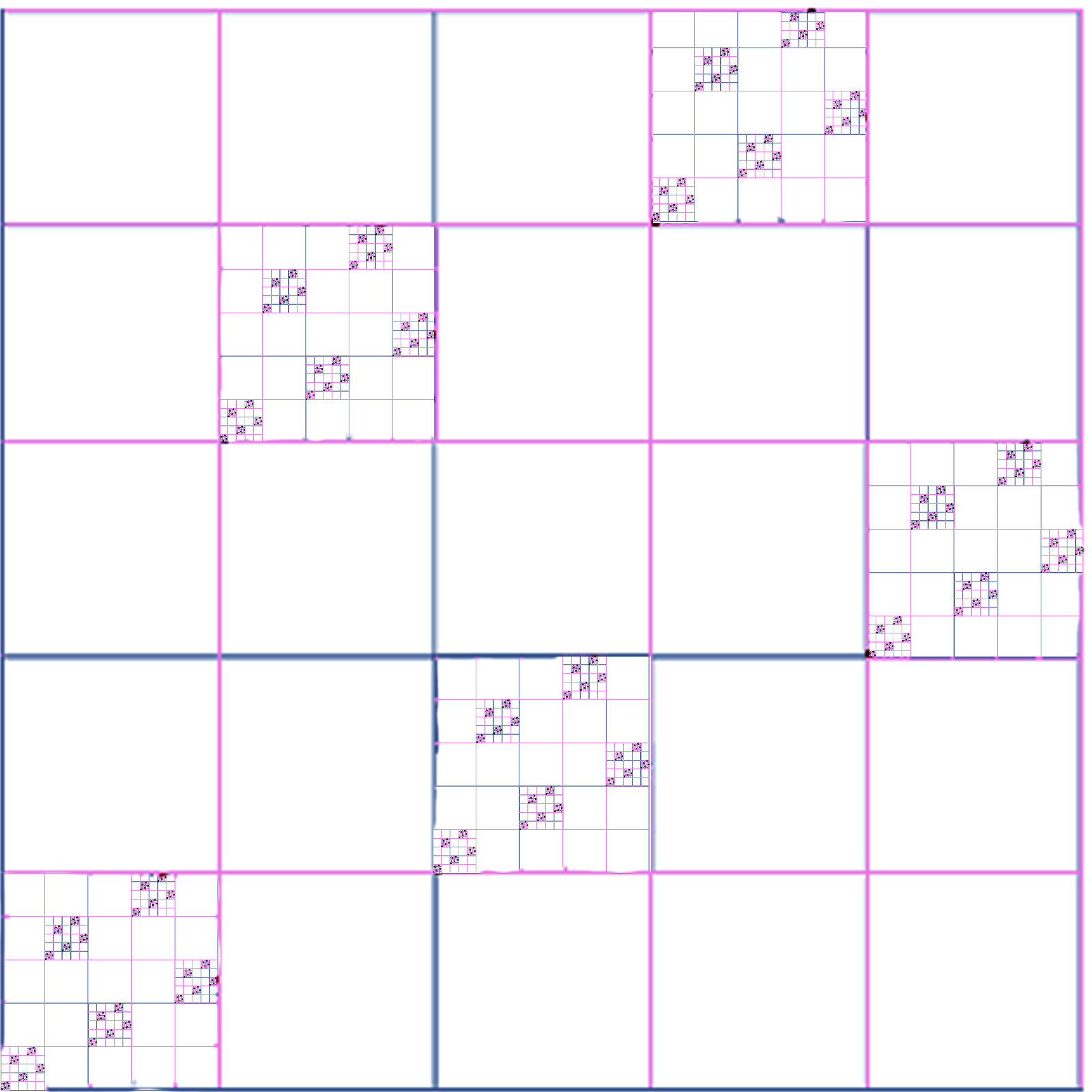## A Chess Puzzle, Part I: The Infinite Chess BoardFirst part of A Mathematical Chess Puzzle:

A while ago I was in the waiting room of the doctor’s office, staring at the ceiling, and looking for something to keep myself amused before the appointment. (The visit was nothing serious, just wanting to update my vaccinations.)

The ceiling was covered with those white acoustic panels, forming a very large colorless and seemingly infinite chess board. I stared at the tiles, trying to think up some kind of puzzle to keep me amused while waiting. I came up with this:

### The Chess Density Problem

Suppose you have an infinite chessboard, and an infinite number of one of the six kinds of chess pieces (King, Queen, Rook, Bishop, Knight, and Pawn). What is the densest packing of “friendly” pieces you can have on that board?

Just asking the question raises more questions:

• What do I mean by “infinite” ?
• What do I mean by “densest” ?
• What do I mean by “friendly” ?
• And how would one prove such things?

So far I’ve spend a fair amount of time and scratch paper on this — quite a bit while sitting around with Gigi’s family for Christmas — and have some things to report. The first version of this post was very math-heavy. Now it is a bit lighter. You’re welcome.

### Disclaimer

Friends who have suffered through games with me will tell you that I am terrible at playing chess, and spend far too long to come up with a bad move that in standard chess notation would probably be denoted “Nf3 Nc6??”. Not just Chess, but Go, Twixt, Bridge or other strategic games. In defense, I can only say that I am spending all of that time trying to solve the problem, based on the game-theoretic fact that a “best” move exists. The decision tree is so deep, however, that no computer yet exists that can explore it completely more than a few moves ahead.

Good chess players acquire skill through experience and study, making decisions with imperfect knowledge. They stand at the edge of the Grand Canyon of moves and can walk down trails blazed by others. I can only stand at the edge, frozen by the awesome infinitude of the depths. Then I fall in.

Anyway, this isn’t the organic chemistry of Chess, but the simpler math of atoms and fundamental particles, Boards and pieces. Moving on…

### Density: A Simple Example

Here is a simple (finite) example of four Queens on a $4 \times 4$ board, where no Queen is attacking any other, either on rank, file, or either diagonal:

So for the $4 \times 4$ board, with its 16 squares, you can only “pack” at most four queens, meaning the “density” (which I denote $\delta$) of Queens on this board is

$$\delta = 4/16 = 1/4$$

Is this the “best” we can do in this case? The answer is yes, for the simple reason that at most one Queen can be on each row (or they would not be friendly), and there are four rows.

This “packing” puzzle is what’s known as a Mathematical Chess Problem, and falls in the category of “recreational mathematics”. That is, mathematics done for amusement rather than “professional” work on “serious” problems. It should be noted, however, that often these problems lead to questions which are actually “deep” and lead to more important work.

In any case, from the literature, the task of arranging “friendly” chess pieces is called an Independence Problem (or an “Unguard” problem), and a chess position in which all of the pieces are friendly is called “Unguarded”. If an unguarded position is the “best” we can do (ie, there is no unguarded position with more pieces), we will call it maximally unguarded.

So, for example, on the standard $8 \times 8$ chessboard, here are examples of how to arrange “friendly” Pawns, Knights and Kings:

We will show (later) that these are “maximally unguarded” positions, and so the density of these pieces on the standard 8-board are 1/2, 1/2, and 1/4, respectively.

And here is how to arrange the remaining three “friendly” pieces, the Rooks, Bishops and Queens:

These last three are maximally unguarded positions, and so the density for these three pieces on the standard board are 1/8, 7/32, and 1/8, respectively.

To see this in the case of the Rooks and the Queens, we can use the same argument as we did above with the Queens on the 4-board, by noting that there are exactly 8 rows and only one Rook or Queen can be on any row.

For the 14 Bishops, you can make a similar argument, by noting two things:

1. There are exactly 15 diagonals in one direction (shown below in blue), and
2. For each diagonal numbered 1 and 15, there is only one square available for placing a Bishop, and those two squares are “hostile” along the red diagonal, so you can only choose one.

We have separated out the pieces into these two sets of three, because each set have their own unique characteristics, unlike the other set; and the differences in density become more pronounced as the size of the boards becomes larger and becomes infinite. Not only that, but the kind of “density” has to be defined differently, and even the size of the “infinite” board becomes important; as we’ll see, some chess boards are more infinite than others.

### Chess Boards: Finite and Infinite

All of the “chess boards” we will be working with will be two-dimensional, and each “square” on which a chess piece can be placed can be indicated by a pair of numbers $(x,y)$, where $x$ and $y$ are coordinates in some set $A$, which we will call the Address space. A chess board that uses coordinates in Address space $A$ will be denoted $B_A$. Mathematically, the board $B_A$ can be identified with the Cartesian product $A \times A$.

For example, the squares of our standard $8 \times 8$ chess board can be specified using $A = \{0,1,2,3,4,5,6,7\}$. The set of integers from 0 to 7 is sometimes denoted $\mathbb{Z}_8$, and so the standard chess board is $B_{\mathbb{Z}_8}$.  To make life simple(r), we will also call this board $B_8$, or simply the “8-Board”, and similarly an $n \times n$ board (where n is an integer) will be called the “n-Board”, or $B_n$.

Here for example is the 5-board $B_5 = B_{\mathbb{Z}_5}$:

Okay, so far so good (you still with me, here?). Now the point of all this mathematical gobbledegook (a scientific term), is that I wanted to look at the “Unguarded” chess problem on an infinite chess board, meaning that I want to use for a “coordinate space” $A$ an infinite set. The first one that comes to mind is the set of whole numbers, ie

$$\mathbb{W} = \{0,1,2,. . .\}$$

And so we can define our first infinite chess board as $B_{\mathbb{W}}$, consisting of all $(x,y)$ where $x$ and $y$ are non-negative integers.

Intuitively, the board can be visualized like this:

This picture looks a lot like the ceiling at my doctor’s office, which is what started this whole thing.

Now the “size” (cardinality) of the whole numbers is “infinite”, but the specific mathematical name for this “infinite” value is $\aleph_0$ (pronounced aleph-null). Unlike the conventional symbol for infinity ($\infty$), $\aleph_0$ has a more precise meaning, and it refers only to “countably” infinite sets, ie, those which you can count with the integers 1,2,3, etc. A related infinite “ordinal” number is called $\omega$ (small omega), and with some “abuse of notation” we will refer to $B_{\mathbb{W}}$ as $B_{\mathbb{\omega}}$, or simply the $\omega\text{-Board}$.

### Chess Boards: Infinity and Beyond

There are many other ways to define infinite boards, but for now the only other board we will look at uses as its coordinate space the closed unit interval on the real line, that is,

$$I = \{ x \in \mathbb{R}: 0 \le x \le 1 \}$$

The board $B_I$ can be visualized as the unit square, including every single point on the boundary and in the interior as a distinct “square” on which you could place a chess piece:

The size of the set of real numbers in $I$ is a transfinite number which is infinitely larger than $\aleph_0$, and is called simply $c$, the infinity of the continuum. If we embrace “The Continuum Hypothesis“, this number c can be identified as $\aleph_1$, the next largest infinity. We shall therefore also call $B_I$ the c-Board, or $B_c$. The number of “squares” on $B_c$, unlike the $\omega\text{-Board}$, is uncountably infinite.

### Sneak Preview

The “density” results for each infinite board and piece will be shown (in later posts) to be as follows.
The pieces are in order of their decreasing (2d or fractal) density, and thus their increasing “power”:

$$\omega\text{-Board}$$

Piece Board 2d-Density Fractal Dimension 1d-Measure (**)
Pawn $B_\omega$ 1/2 2 *
Knight $B_\omega$ 1/2 2 *
King $B_\omega$ 1/4 2 *
Rook $B_\omega$ 0 * *
Bishop $B_\omega$ 0 * *
Queen $B_\omega$ 0 * *

$$c\text{-Board}$$

Piece Board 2d-Density Fractal Dimension 1d-Measure (**)
Pawn $B_c$ * * *
Knight $B_c$ * * *
King $B_c$ * * *
Bishop $B_c$ $0$ $1$ $2\sqrt 2$
Rook $B_c$ $0$ $1$ $2$
Queen $B_c$ $0$ ? ?

(*) Not Defined for this piece on this board.

(?) Unknown whether a Hausdorff-measurable solution exists.

(**) 1d-Measure = (Hausdorff Content)

As you can see from the two tables, there is something very different between the two groups of pieces, and how they “pack” on those infinite boards. In following posts, I will try to explain what the differences are, what a Fractal Dimension is, and what other mathematical issues and questions arise in the process.

## Longitude Part I: The Geometry of a Sextant## The PurchaseAs I was only wanting to acquire a sextant for our book club discussion on Dava Sobel’s book “Longitude” (see below), I didn’t want to spend much money, and so put down $15 on Amazon for the brass sextant you see pictured above. I was hoping that the thing would be functional enough that I could demonstrate its usage, but found that not to be the case, as-is. I suspect that this object I bought was not so much a sextant, as a knock-off of a copy of a replica of a sculpture of an artist’s rendition of a sextant. I have now formed a vague notion that this item was produced in a back-alley of a run down area of Calcutta or Shanghai, by a person with little education but some skill in metalworking, casting, and possibly jewelry. Whether they have ever been on a boat, or could pick out the star Regulus on a clear night (city lights permitting), the question remains open. One way or another, the good news is that after realizing this object was not functional, in the process of making it so I found that I learned a lot more about sextants that I ever would have, had I bought a truly functional precision instrument (for$200 more) in the first place.

So let’s get to work.

## The Sextant

Though they look complicated, the simple idea behind a sextant (or quadrant, octant etc) is just to measure the angle between two things in the sky, either two bodies (like the moon and Regulus), or between one body (Polaris or the sun) and the horizon. This is easy to do on land, but at sea with everything moving it is difficult.

The clever idea (which it appears Newton had first) is to use two mirrors (actually, one and a half), in such a way that the two objects you are measuring can be brought “next” to each other optically by adjusting one of the mirrors. Once done, it is then just a matter of precisely measuring the angle the movable mirror was rotated. This nice diagram below (gleefully stolen from Wikipedia) shows how to get the (elevation) angle of the sun above the horizon:

While we are on the topic, we should show the proper names of all the main components of the sextant:The main elements are the frame, which is the 60 degree wedge that forms the base of the sextant. There is usually a handle on the other side of the frame so you can hold it. Along the outside of the frame is the arc, which has degree markings, starting from zero on the right. The frame also holds the fixed “horizon mirror“, which is only half-mirror, half clear glass. The movable arm is the index bar, which has a pointer (the index) that points to the angle on the arc. The “index mirror” is fixed to the index arm, and is a full mirror that rotates on a pivot and brings the second object into view. The shade glasses are deployed for shooting the sun, and prevent you from going blind. The drum allows you to do fine adjustment of the index arm, which whose angle on the arc you can see with the magnifying glass. Finally, the telescope is a tiny low-powered telescope which allows you to get a good look at the objects whose angle you are measuring.

Here is an oblique view of my sextant, lying on its side. Ordinarily the geared arc is pointing down toward the earth. You can see that the horizon mirror is clear on the left side, and mirrored only on the right. So when you hold the sextant with the the telescope pointing to the horizon, the left side is looking straight ahead, at the horizon. Meanwhile, the right side is reflecting light from the index mirror, which is coming in at some angle above the horizon (indicated by the index arm).Here for example is the sextant with the index arm angle set to zero. This setting should allow the light from the horizon to bounce off the two mirrors and come into the little telescope at zero degrees. In other words, the view in both the left and right half of the horizon mirror should match.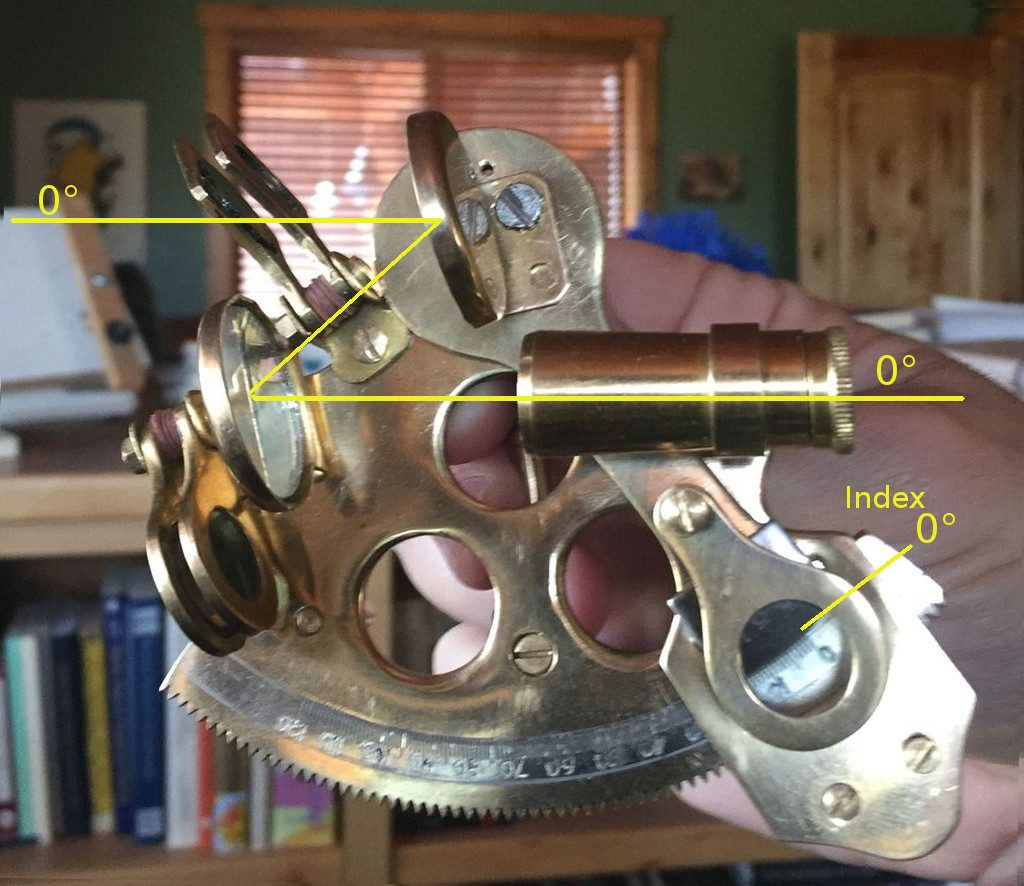## The Geometry

The first geometrical question to address is, how does changing the angle θ of the index arm affect the angle β of the light coming in from the index mirror? Intuition suggests that since there are two mirrors, the angle will be doubled. Indeed, the rule is:

 The angles on the arc of the frame should be marked like a protractor, but with the angular values doubled.

Of course (for me) this requires proof. We will need to draw a diagram: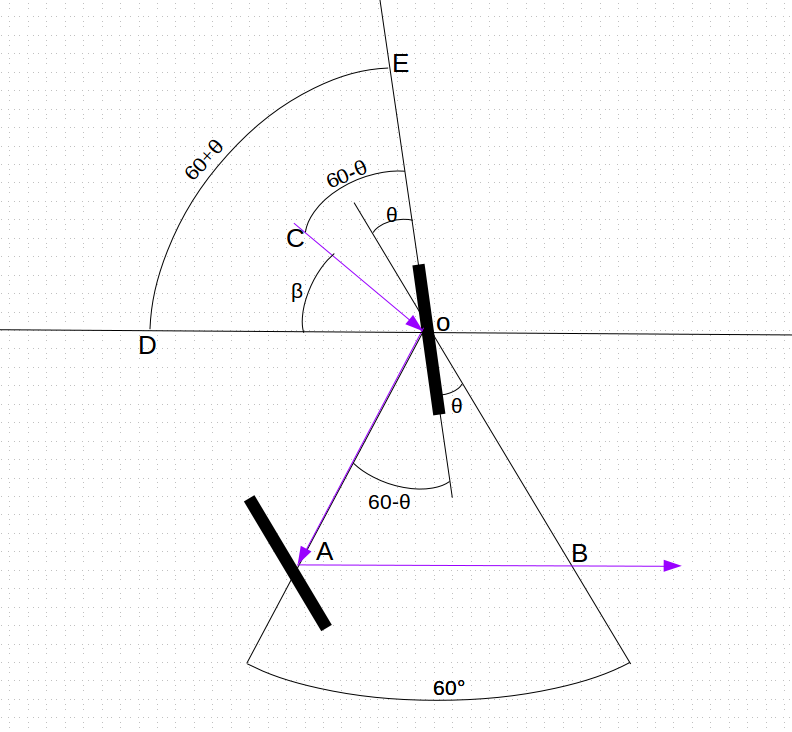The lines in blue show the path of light coming in along line CO, reflecting off the index mirror at O, continuing along line OA, and then reflecting off the horizon mirror at A, finishing along AB to the telescope. Let’s assume the index mirror makes an angle of θ with the line OB, and so the angle between the ray of light OA with the mirror  at O must be 60°-θ. Now light bounces off of mirrors at the same angle they came in, so the incoming ray of light CO must also form the angle COE which is equal to 60°-θ. Finally, the index mirror forms an angle EOD with the horizontal line OD of 60° + θ, meaning that the residual angle β we seek is the angle EOD minus EOC, that is,

β = EOD – EOC =  (60° + θ) – (60° – θ) = 2θ

so β = 2θ. That is, the angles marked on the arc, in order to properly represent the incoming angle β of light on the index mirror, must be a value of exactly twice the actual angle formed by the index arm at that point from the zero mark.

## The Reformation

It didn’t take long for me to discover that my shiny new 15 sextant was not really functional as-is. It needed work. So the first thing I did of course (as is my nature) was to take the whole darn thing apart.### Issue #1: Frame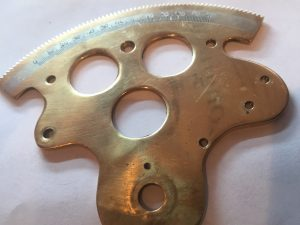The largest and most important component is the Frame — the large flat bit with the round gear-teeth around the edge. This serves as the “optical bench” upon which all the components are mounted. In addition, the gearing must be uniform, and the markings on the Arc calibrated, so that precise angular measurements can be made. The first problem was that the frame was not flat. As many of the components are mirrors which must be aligned in 3 dimensions, the subtle bends in the frame would throw off any measurement. With all the pieces now removed, I was able to flatten out the frame. The second problem was that the markings on the arc were clearly not precise. One clue may be found in the numbers, which on close inspection seem to have been crudely carved in by hand with a Dremel or similar tool. This is not a problem that can be resolved, short of recasting the whole thing.### Issue #2: The Index Mirror The first thing to note about the correctly made sextants in the previous section is that the index mirror is not aligned with the axis of the index arm, but slightly off, about 15 degrees. The one I got by comparison was not like that, and its mirror was lined up exactly with the index arm like this: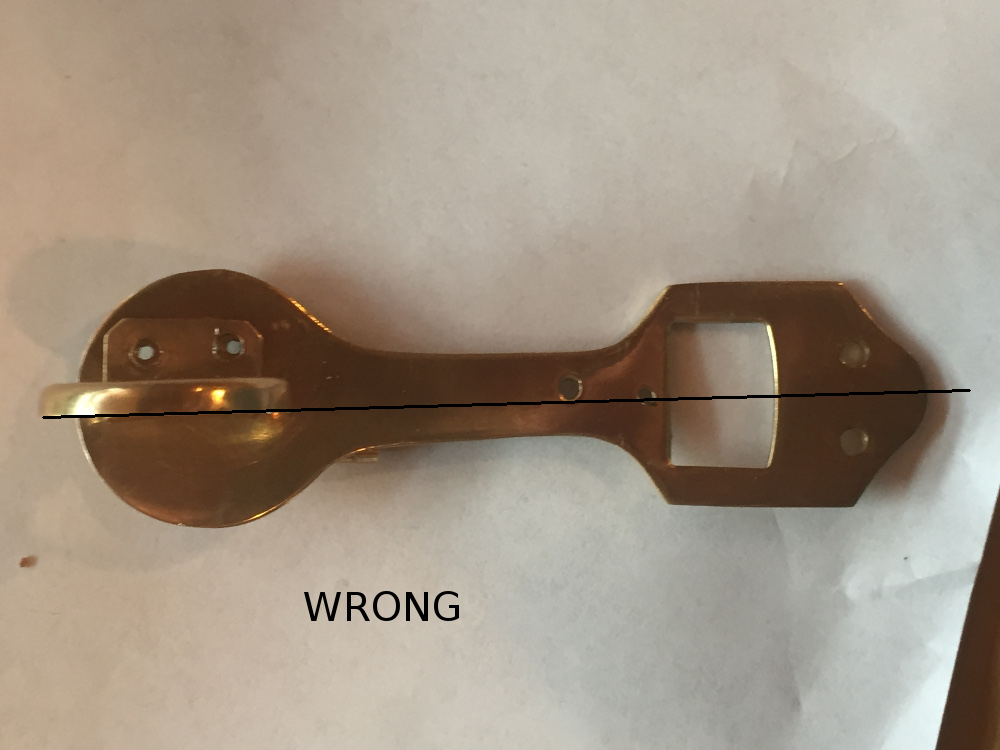It turns out that this is wrong, or at least not very good or practical design. In fact, what it should look like is this: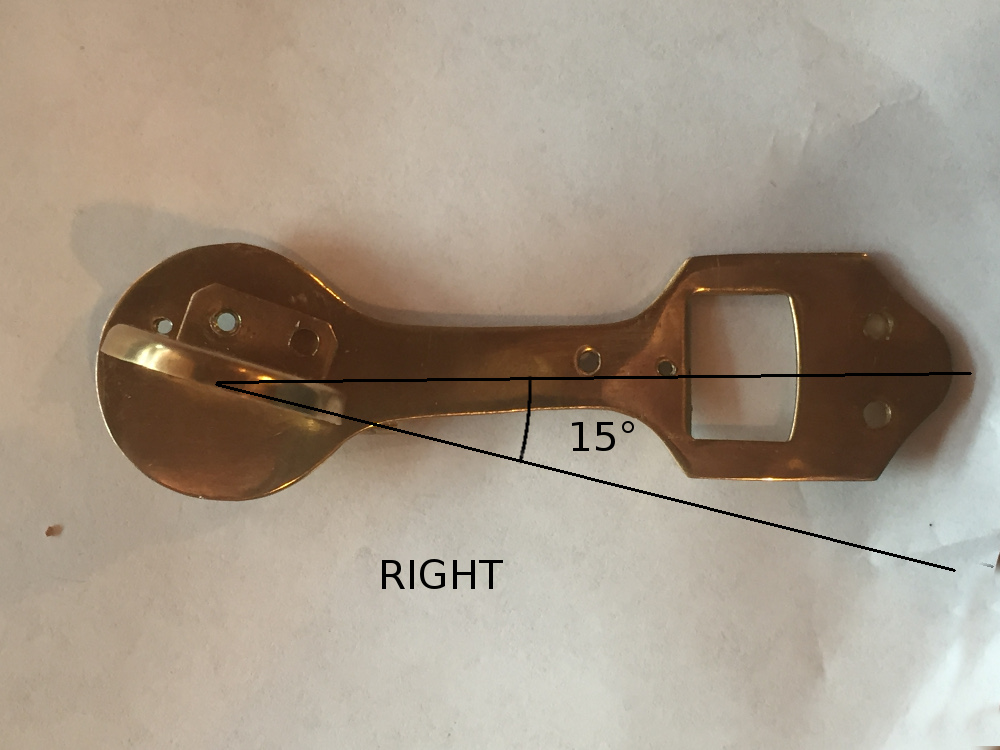Now the actual angle does not need to be 15°, but should be around there. The rule here is a bit more heuristic and goes like this:  The index mirror should not be in line with the index arm, but offset by an amount greater than zero but less than 30°. 15° is close to ideal. So what’s going on here? This is a mixture of mathematics and practical engineering. Let’s parameterize this situation, and define a sextant whose index mirror is off-axis by an angle of δ a δ-Sextant. By this definition, what I bought is a 0°-Sextant, while the ones in the cartoon diagrams appear to be 15°-Sextants. Okay, so what is so bad about my 0°-Sextant? Well, for looking at objects near the horizon (ie, where the index arm is near 0°), there is nothing really wrong at all and it works fine. I’m not familiar with the original design considerations, but two big factors have to do with the positioning of the horizon mirror, and the light-gathering ability of the index mirror. So let’s consider the horizon mirror first. Here is the geometry of the general δ-Sextant, with the index arm set at zero (so we are looking at the horizon):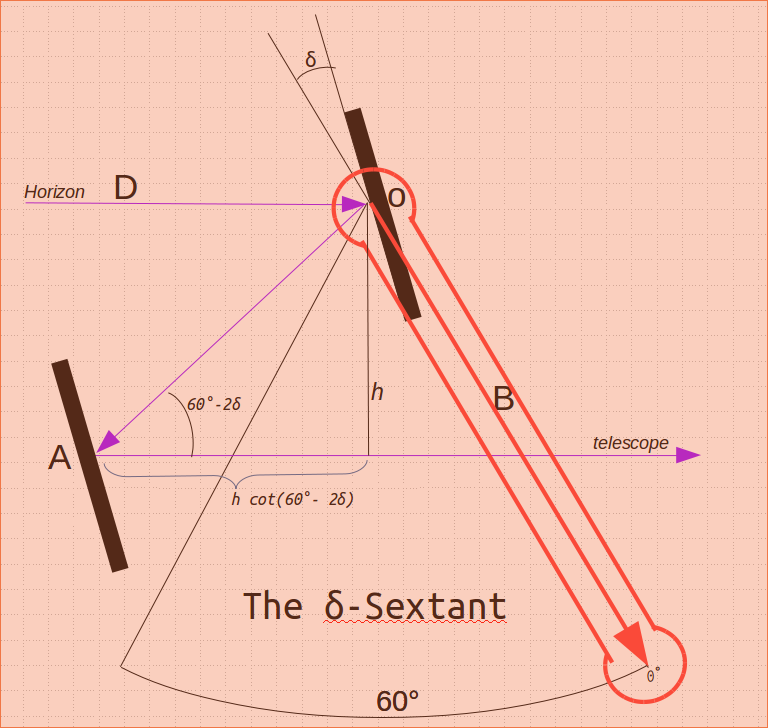Now by the same argument as before, the successive reflections of the ray of light coming in from the horizon form an angle of 60° -2δ away from the horizontal. So in order to the light to drop down a height of h (so that it can reach the telescope), the horizon mirror must be set back by h*cot(60° -2δ) from the center of the sextant. As we increase δ from 0 to 30 the cotangent approaches infinity, making the mirror position increasingly impractical. Therefore, taking a mid-point value of δ=15° would put the mirror in a practical location, much less than infinity. The mirror was mounted on the index arm with two small screws. I drilled two new holes for the screws and used a tap-and-die kit to thread the holes for the screws. The new mount for the mirror brought it close to the required 15 degree orientation. ### Issue #3: Index Gear “Drum” The index gear “drum” as depicted in the good diagram is a very precise worm-and-gear arrangement, with a “micrometer” fine-tuning for getting fractional degree measurements.The knob is supposed to engage the gear teeth in the frame, and rotate the movable index arm (on which the index mirror is mounted). However, instead of using a worm-and-gear mechanism, it has a “direct drive”, in which the knob turns a wheel gear that meshes with the frame.On close inspection, it was found that this gear must have been made by drilling out each of the gear teeth, and manually filing them down. The width and separation of the teeth have a visibly discernable variation, of about 5% or so. This bit is of the “juggling dog” variety, which is to say the amazing thing is not that it does its job well, but that it does it at all. Indeed, this gear tended to jam up at certain parts of the arc, and so required some additional filing just to get it to juggle at all. ### Issue #4: Vernier Scale In place of the high-precision worm-gear/micrometer, this sextant uses a “Vernier Scale“, which is admittedly a very clever device that was invented in ancient China (but named after French mathematician Pierre Vernier) to extract more precision out of otherwise crude devices. The general idea is to have a second scale that is just slightly smaller (9/10ths) than the base scale. This makes the smaller scale lines rarely line up with the base scale, except at one marking, which indicates how many tenths of a marking need to be added to the base reading: Here is what our vernier scale looks like (bolted to the index arm):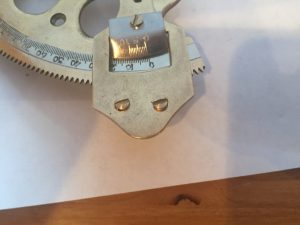There is just one problem with our Vernier. The scale is not 9/10’s of the base, but 10/10ths: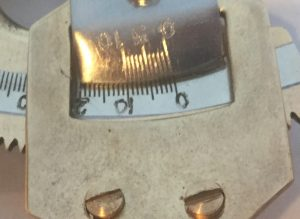In other words, as a Vernier scale, it is totally useless. It is most likely that the poor starving artisan in Bangalore was just told to copy another copy and assumed the scales were supposed to match. There is no way to fix this. But as the gears that drive the index arm along the arc are themselves only accurate to about 5%, the additional precision that would be provided by the Vernier is pointless. At least it has a 0 degree line, with which I can line up the 0 on the arc and calibrate zero-degree separations. ### Issue #5: Sun Filters While sextants are often used at night to measure distances between stars, they are also used to calculate the Sun’s altitude above the horizon (at noon for example). For this reason, sextants come with sun filters to prevent injury to the eye. The sun filters on this sextant are faintly colored glass, and should NEVER be used for any reason. Hopefully, nobody ever has used them. They are, like the rest of the sextant, purely decorative. The only fix is to replace with actual solar filters, or remove them altogether.### Issue #6: Telescope To its credit, the telescope is not really a problem. It even appears to have a very slight magnification, and is properly aligned with the fixed horizon mirror. ### Issue #7: The horizon mirror The horizon mirror, ideally, is split down the middle, with only the right half mirrored and the left half transparent, allowing the direct forward view to come through. Unfortunately, the mirror was glued in slightly at an angle, so that the vertical line between the mirrored and transparent sides is tilted by about 5 degrees. I did not want to risk breaking the mirror so left it alone. It was also offset vertically from the index mirror, and so added spacers to raise it up a bit.### Issue #8: The Handle and Horizon bars The back of the sextant has a vertically oriented handle, along with two posts which when aligned should match the horizon (when computing the sun’s altitude):The vertical handle was not exactly vertical, and so I inserted a spacer to adjust the alignment:### Issue #9: Magnifying lens The magnifying lens, intended to magnify the vernier and base scales for easy reading of the angle, doesn’t magnify. It appears to be an optically flat piece of glass, identical to the “sun filters”. I removed it as it only gets in the way. ## Conclusion This was a pretty but functionally useless toy when it arrived. After two weeks and a number of visits to Ace Hardware, it was almost serviceable enough to calculate separations to about 1 degree. I would not depend on it to save my life. ## The Planet Uranus in OppositionNote: I hope to update this piece when I catch Uranus at the turning point, August 2018. As of right now we are in thunderstorms, so it may take a while. ## Okay Let’s Cut to the Chase Here is an animated GIF of two astrophotographs I took of the planet Uranus from my house in Virgin, Utah. The first one was taken on October 24, 2017 around 2:00 am, the next on November 19,2017 at 10pm (click on the image for full-size animated versions). See if you can spot Uranus. In the course of that month it has moved a bit, near the center of the image, so you should be able to see a blue-green dot jumping back and forth. If you still can’t catch it, here is an annotated versions, with labels and stuff (again, click on the images for the full screen version):In addition to the dated labels, I have put in some graphics showing the constellation Pisces, as well as a chart, showing where computer models say Uranus should be in that part of the sky, for various dates between 2016 and 2020. I had to pull all of these other things in, just to convince myself that I really caught the planet, and not just a random earth satellite or other transient object. It has taken me quite a bit of work to get to this final product, of which I am quite proud, and happy that it came out so cleanly, riding exactly along those predicted lines. In August of 2018 (now) I hope to capture that endpoint of maximum extent. The rest of this blog piece is the retelling of the story of this image, along with the occasional digressions into the geometry of the whole thing. ## About the Planet Uranus Here is a picture of the planet Uranus, taken by the Voyager II spacecraft in 1986. By the time I came to work at the NASA Jet Propulsion labs in ’87 the Voyager II probe had already passed by Uranus and was approaching Neptune, so I never got a chance to see these “live” images coming in. It’s not much to look at, and is best described as a large ice ball (unlike Saturn or Jupiter which are mostly gaseous). Even with a really good earthbound 8″ telescope, you’re not going to see much more than a fuzzy dot. Though it had been seen before (even in ancient times), the object was not identified as a planet until it was observed and reported by William Herschel in 1781, who thought it might be a comet. However, after reporting it to the Astronomer Royal Nevil Maskelyne (who figures prominently in the quest to measure Longitude), Maskelyne concluded that it was probably a planet. Other than its name (being the only one in the solar system based on the original Greek gods, and not the later Latin names of the Roman gods), Uranus is notable for having its rotational axis nearly horizontal to the orbital plane, so that for half the Uranian year (about 45 earth years) the “north” pole is in perpetual day, and the other half the year is perpetual night. ## Uranus in Opposition What started all this for me was the announcement last month that the planet Uranus was in what they call “opposition“, meaning that it was on the opposite side of the celestial sphere (as seen from Earth) as the Sun. From the Sun’s perspective, this means that the Earth and Uranus are on the same side of the Sun, and typically on closest approach to each other: The news in social media suggested that it would be so close that “it could be seen with the naked eye.” That sounded like hogwash to me, as there have been a lot of viral bogus memes around about being able to see things like the rings of Saturn and such. Having now tracked down the planet, I can attest that — technically — it would be possible for a young person with excellent sharp eyesight to see the planet Uranus without binoculars … if they knew exactly where to look, and gazed at it out of the corner of their eye, and in a place (like where I live) with extremely dark skies and no cities nearby, but only on a cool clear night. But otherwise, forget about it. ## The Plan Anyway, with the announcement of the opposition of Uranus in October I decided that this was a good opportunity to do some amateur astronomy and try to capture Uranus with some very low-tech equipment, which is a Nikon D-5000 camera mounted on a crude “Barn Door” equatorial mount. Using this mount, I can take long-exposures of up to 15 or 20 minutes, without smearing of the stars due to earth’s rotation. The Barn Door mount is a clever contraption which anybody can build with20 of parts from Ace Hardware or Walmart. The idea is simple, you just have two boards attached with a hinge, one board fixed to a tripod. You line up the hinge with Polaris (the north start), and mount the camera to the board that moves.

But before setting up my rig, I first had to track down the current position of the planet, which was said to be somewhere inside the constellation Pisces (the fish). Credit here must be given to Martin J Powell’s website NakedEyePlanets.com,  which has this great chart of the path of Uranus:## Navigating the Stars

For anyone interested in astronomy, one way to begin is to learn how to find your way around the sky visible to the naked eye, without aid of telescopes and such. To do this, you need to learn some old-school tricks, in the form of stories. For example, to find Polaris, you first find the Big Dipper (Ursa Major) and follow the line traced by two of the stars in the “pan” of the dipper.

Rant: With the latest GPS-enabled telescopes, it is far too easy to track down stars, planets and other bodies. These days, all you need to do is type in the name of the object (e.g. Uranus), and the telescope’s computer will use its GPS to determine where the telescope itself is located, as well as the current date/time, and then guide the telescope to the place in the sky where the object may be found. Or you can use one of the “Planets” apps on smart phones, which you can hold up in the sky and see what you are looking at.

## Relativity, Simplified. Really.I’ll make this (mercifully) short.

Consider this statement, which I call Ω:

 Everything travels through the Cosmos at exactly the speed of light.

Ω is Relativity. That’s it. Really, it is. And Ω is true not only for Special Relativity, but the General one too. All you have to know is that the “Cosmos” means both space and time.

If you were to ask somebody what Relativity says, they would probably say something like “everything is relative.” The problem with that is, it’s not precise. In fact, it’s not even true. Some things in physics (and the world) are thought to be absolute. So I’ve been trying to come up with a good version of Relativity that can be justified mathematically, and that little box is what I came up with. If you understand what each word means exactly, everything follows from this statement, and if you like you can stop reading now and get on with life. Because that really is what relativity says.

The rest of this short post is just me rambling about why I like it. In a later post I’ll defend it. Also, here is a cool picture of a galaxy for no reason. But it’s cool (*).

### Why I like This Version of Relativity

One of the things I like about this version (Ω) is that it is simple and precise, sounds a little strange — but just enough strange to be right — and answers immediately a number of other questions people ask about relativity, matter, speeds etc. For example:

Q: Will we ever be able to go faster than light?

A: NO. The reason you can’t go faster than the speed of light is that you can’t go any slower either. Nothing in the universe can go any speed in spacetime but c, the speed of light. The only thing you can ever change is the direction in space-time that you are going.

Q: Why does Relativity say that when you go faster, time slows down?

A: Because you are always going exactly at the speed c, so if you go faster in the space directions, you have to go slower in the time direction so it still adds up to exactly c.

See?  Details later, film (and math) at 11. But really, it is true. Ask a physicist. He’ll scratch his head, then say yeah, that works, then go have a beer.

(*) Photo: (Image Credit: X-ray: NASA/CXC/CfA/R.Kraft et al.; Submillimeter: MPIfR/ESO/APEX/A.Weiss et al.; Optical: ESO/WFI)